# Selina Solutions Concise Physics Class 10 Chapter 1 Force

Selina Solutions Concise Physics Class 10 Chapter 1 Force provides students with accurate solutions for all the questions mentioned in the Class 10 textbook published by Selina, as per the ICSE. These solutions are crafted by the experts in the subject of Physics. Selina Solutions are provided in lucid and logical language for students to understand them thoroughly. Selina solutions also help students to analyse their performance while solving.

Students of Class 10 can go through ICSE Class 10 Physics Selina Solutions to clear their doubts which arise while practising exercise questions of Selina Physics textbook. Solutions are well structured according to ICSE guidelines, in an understandable language which enables students to learn the covered concepts with ease.

Smart study is important in scoring satisfactory marks. Students can grasp useful tips and tricks from the Selina solutions, thereby enabling them to perform better in examinations.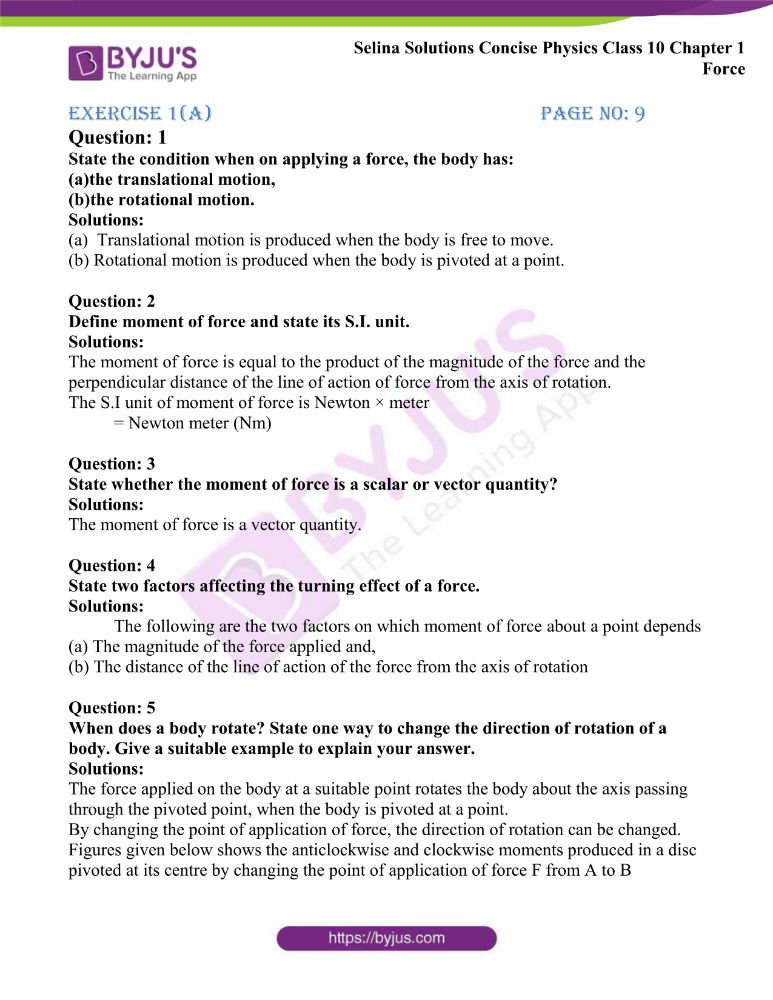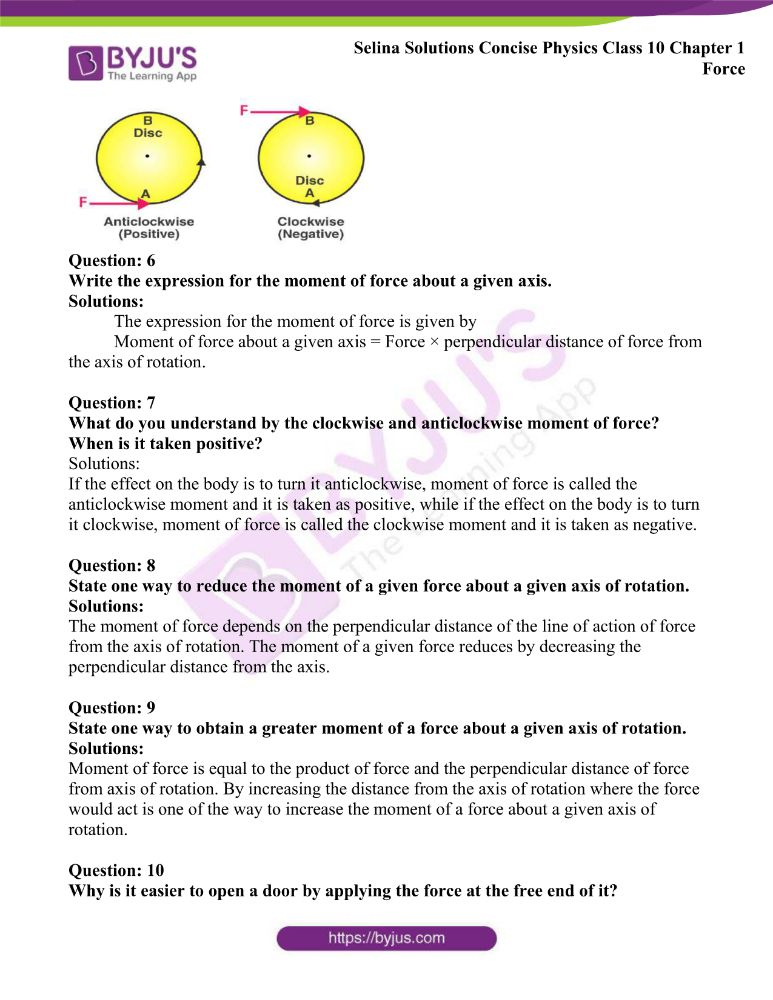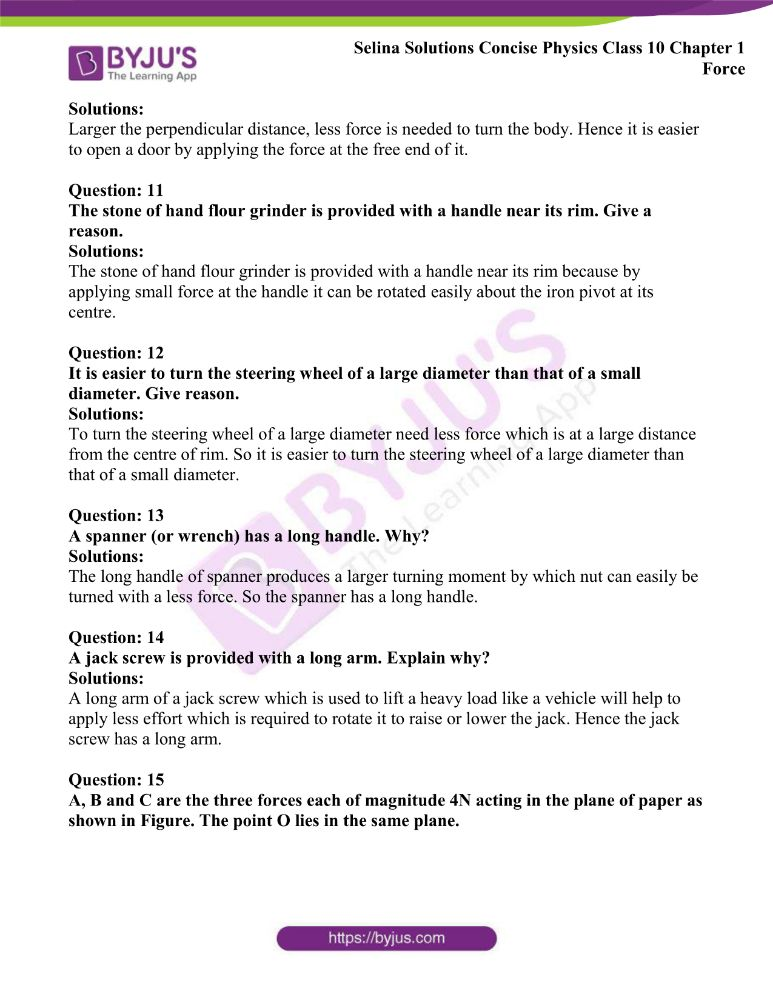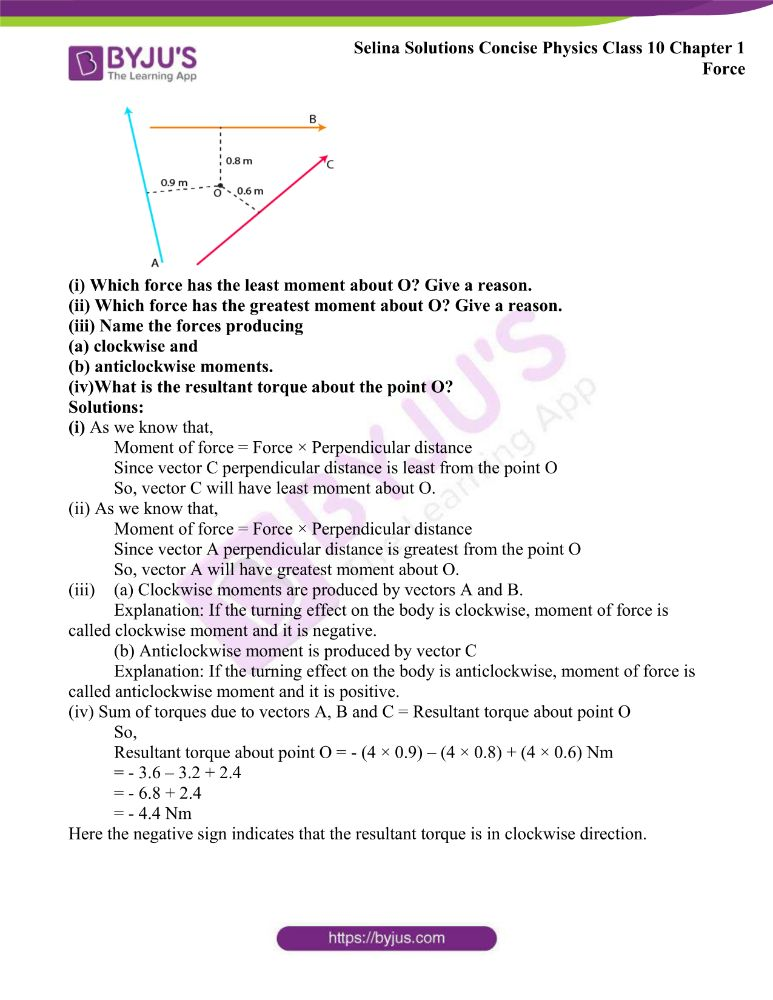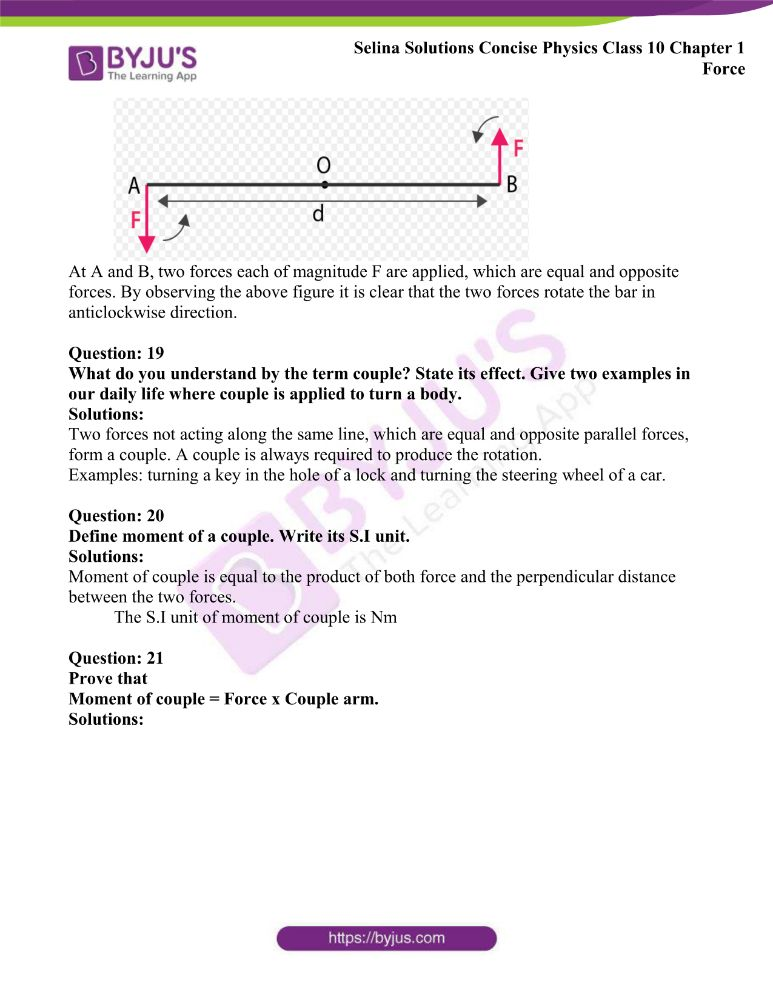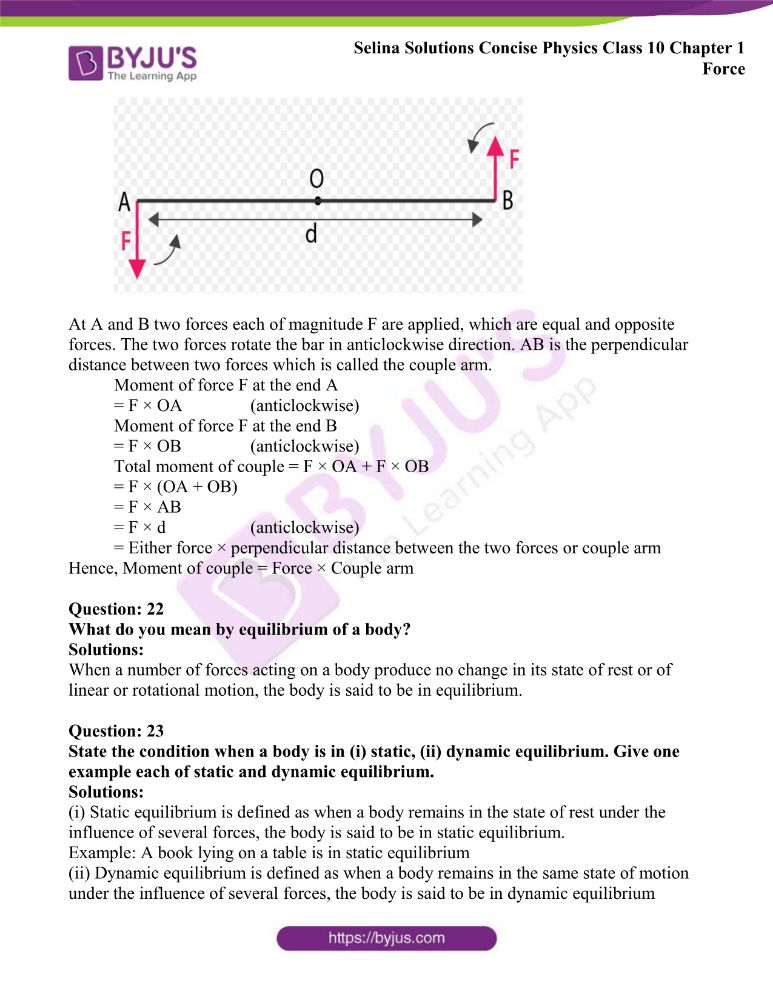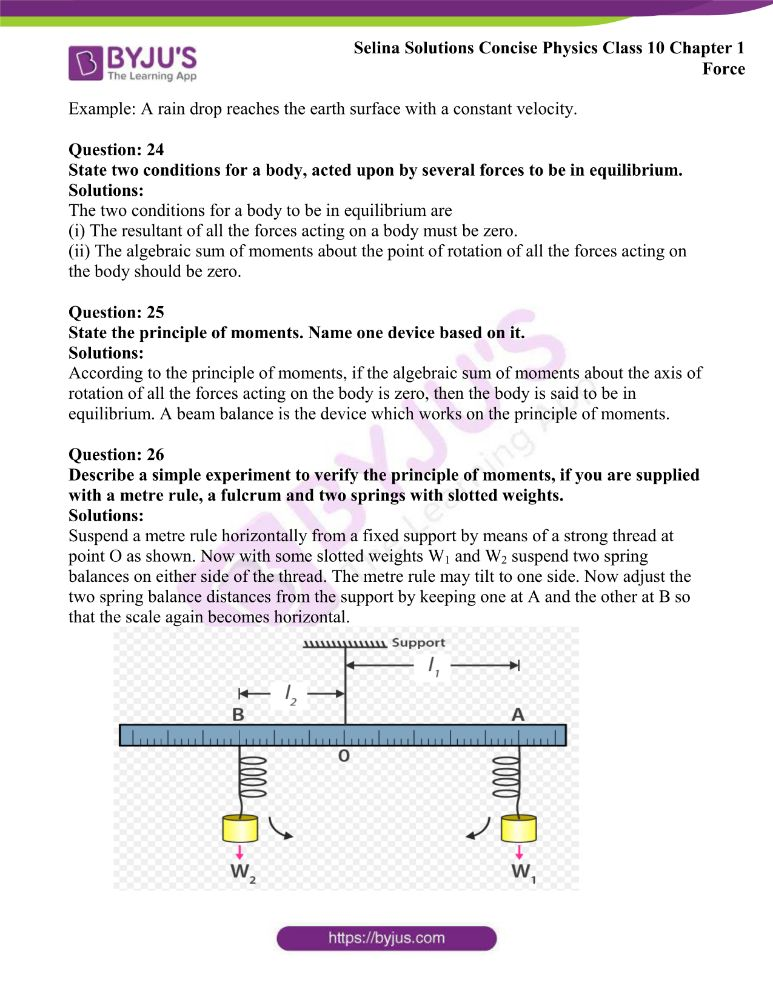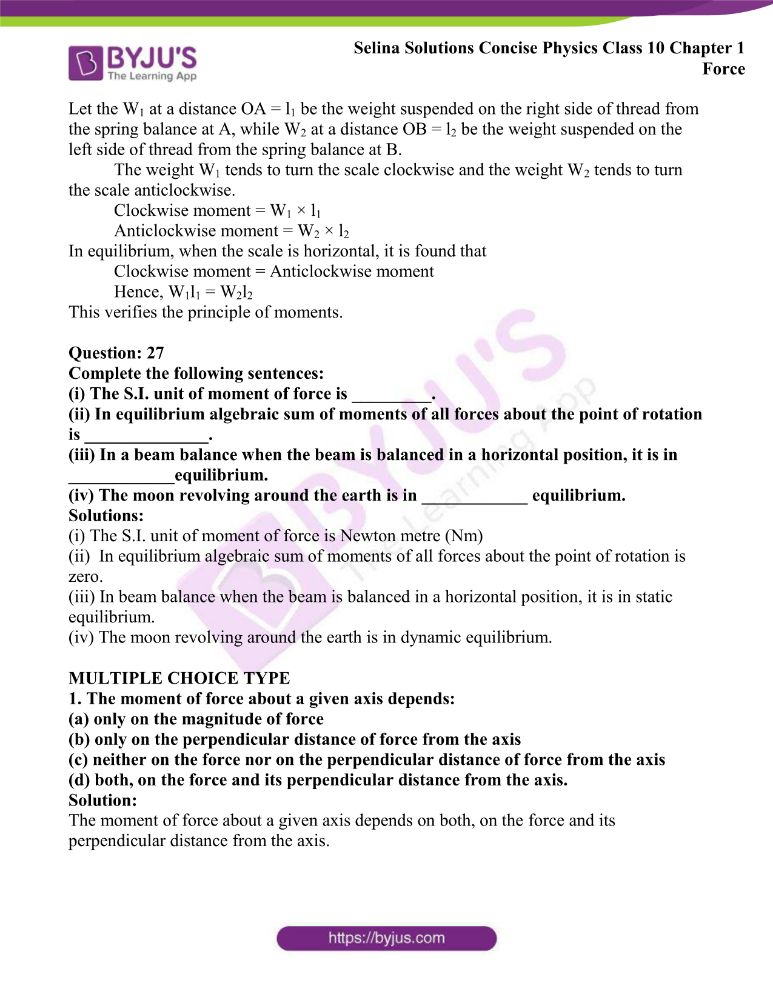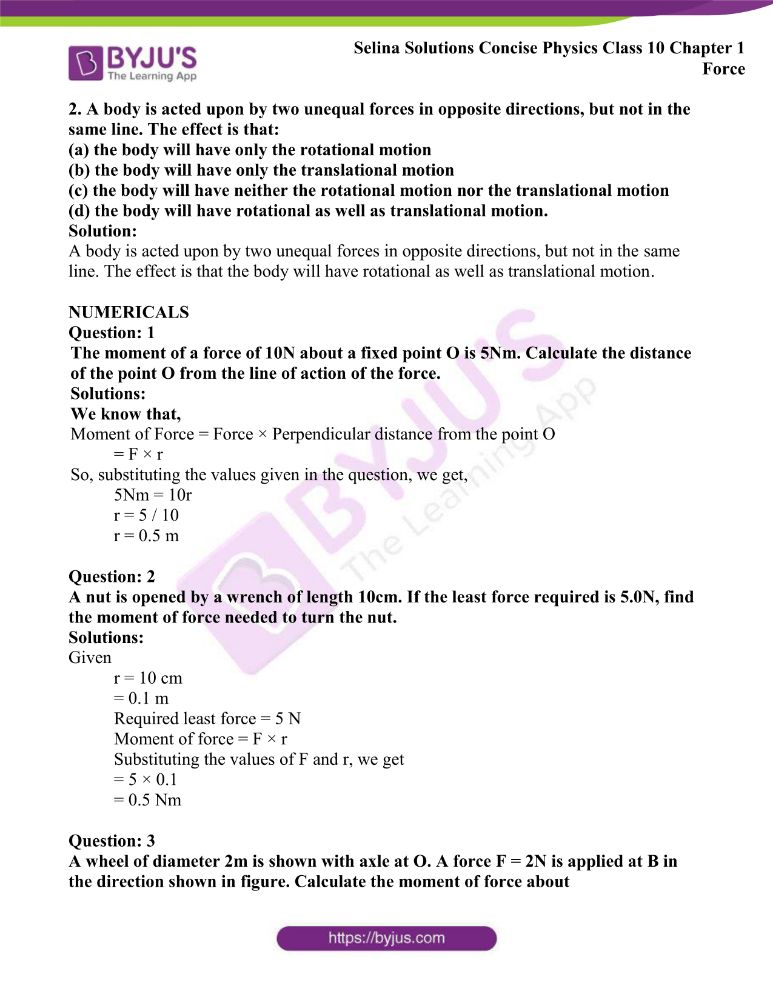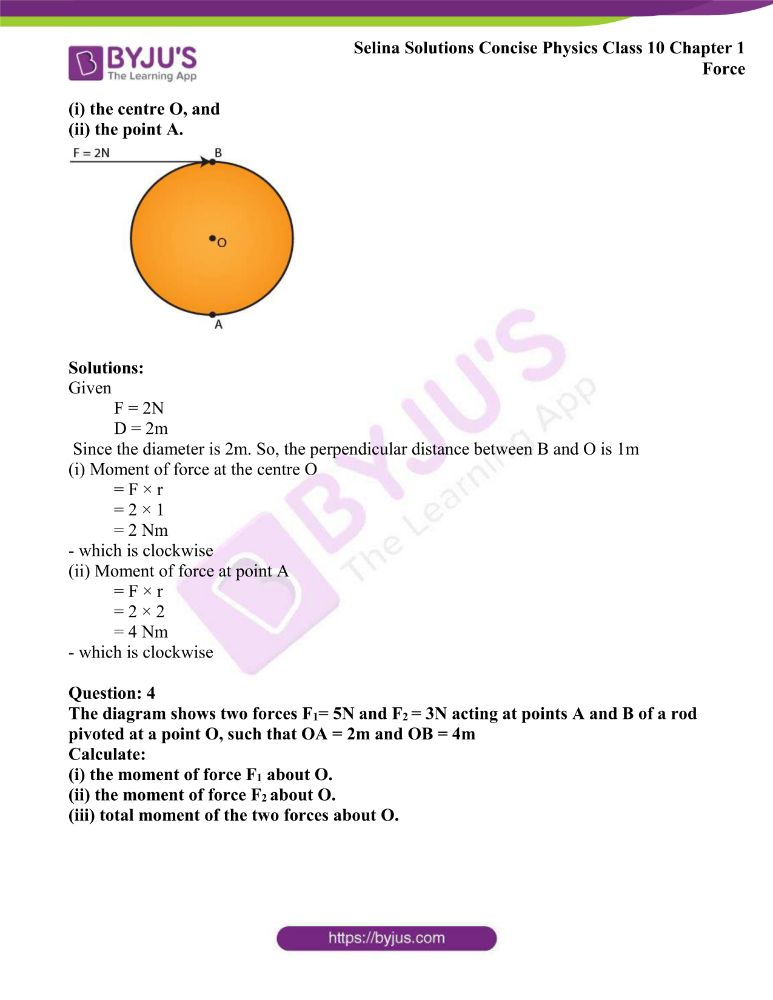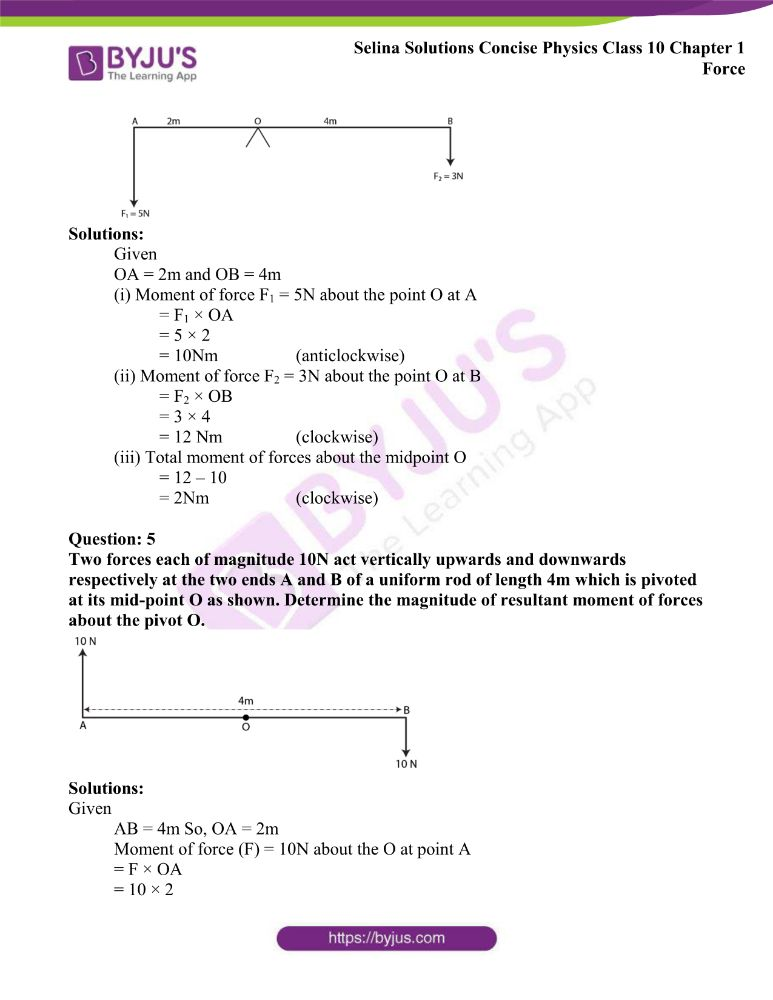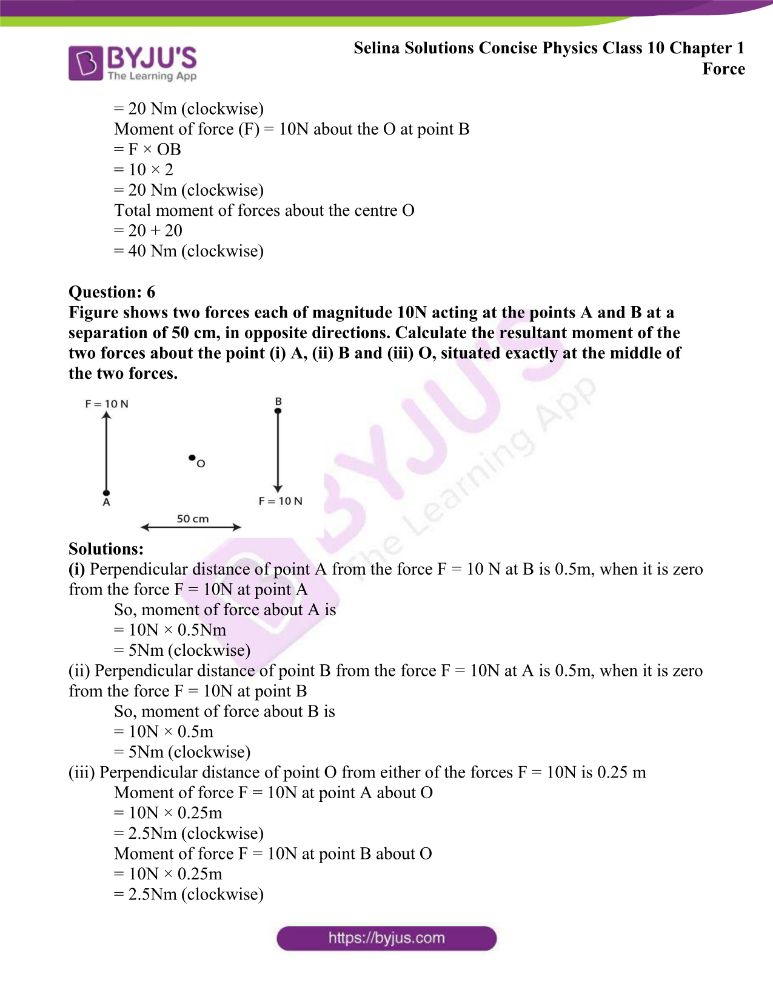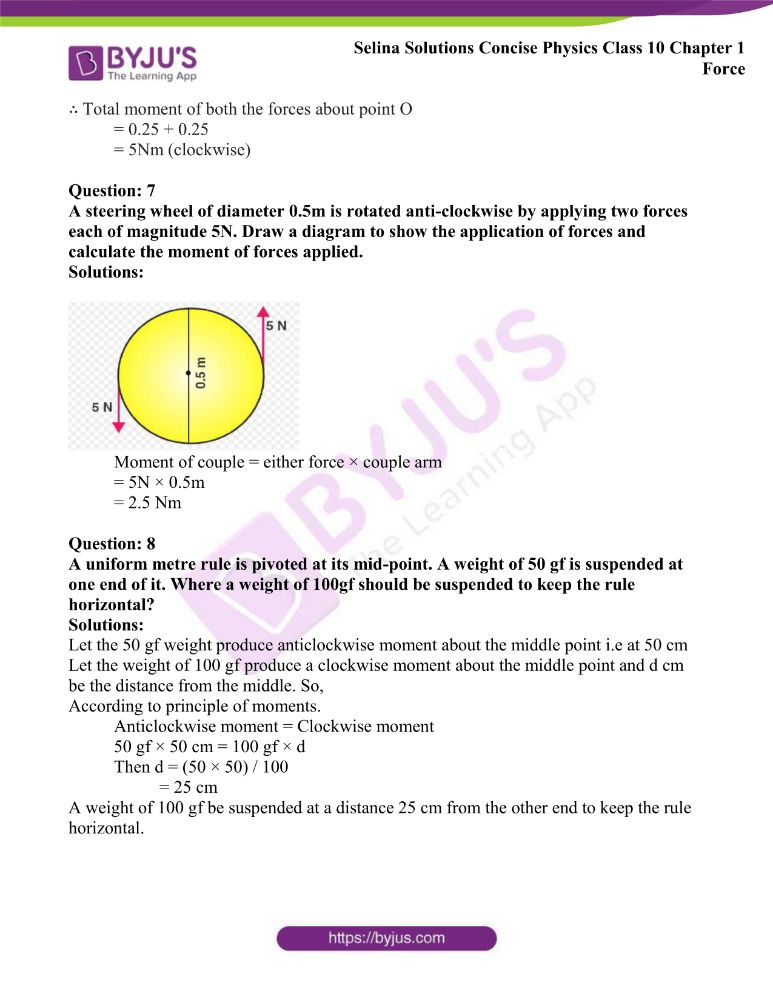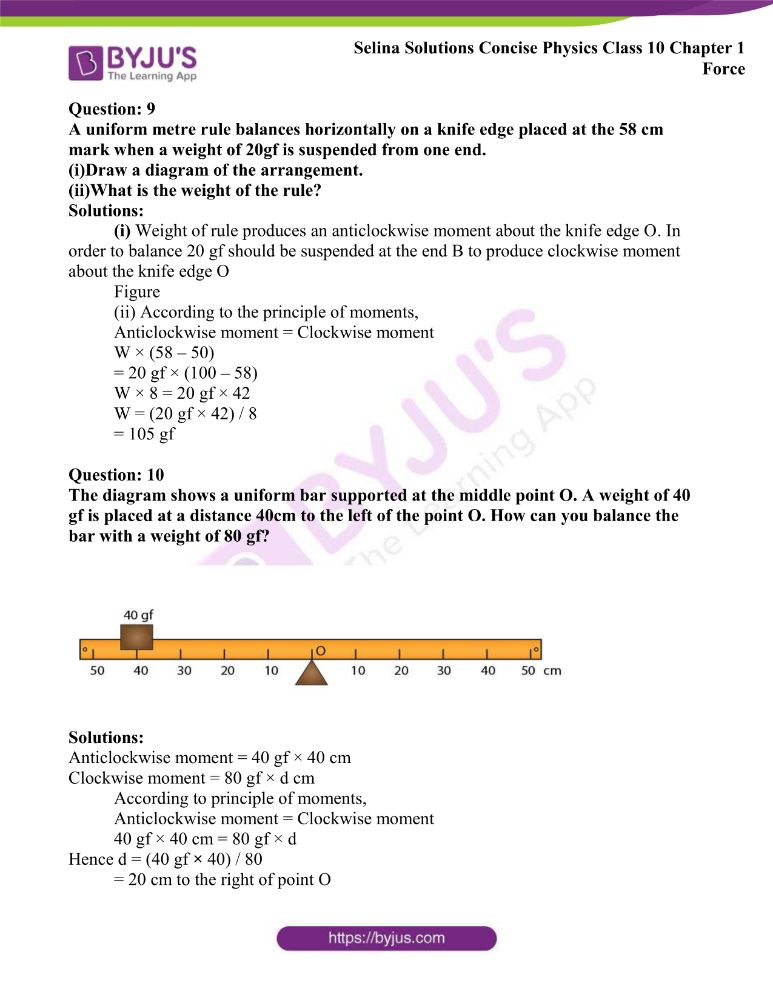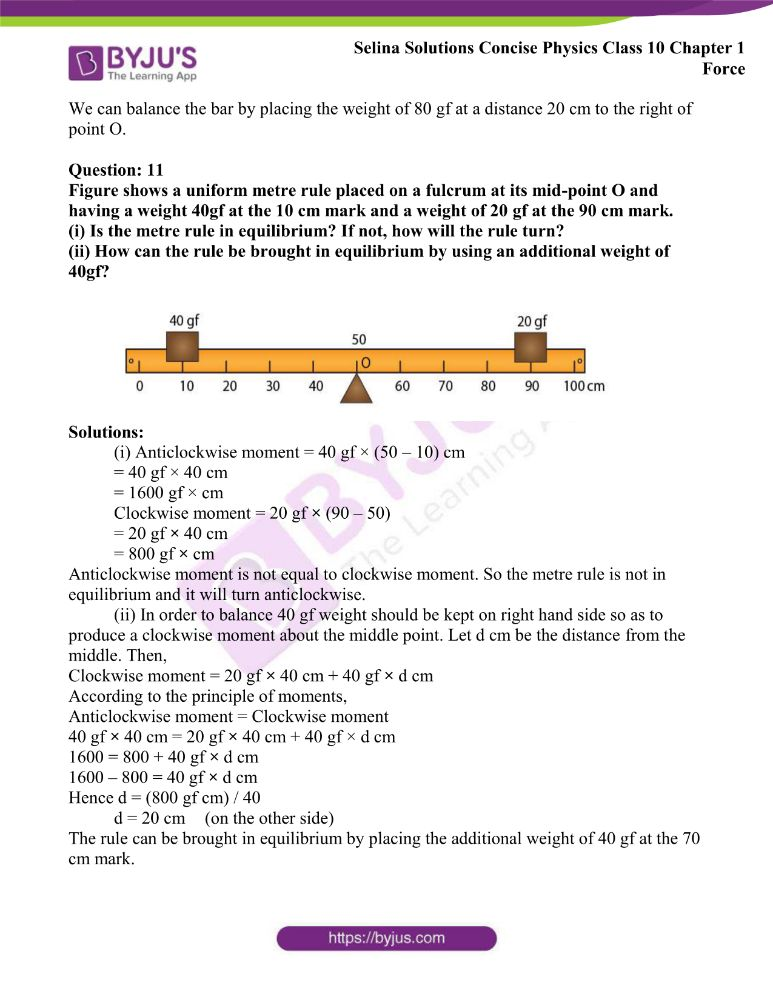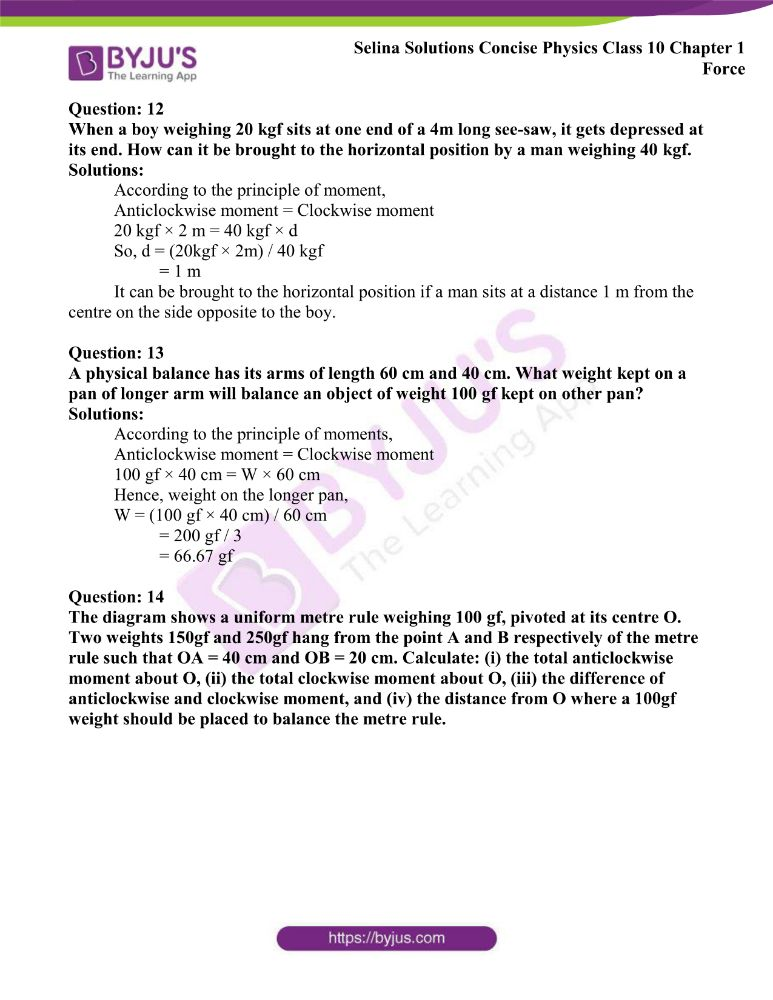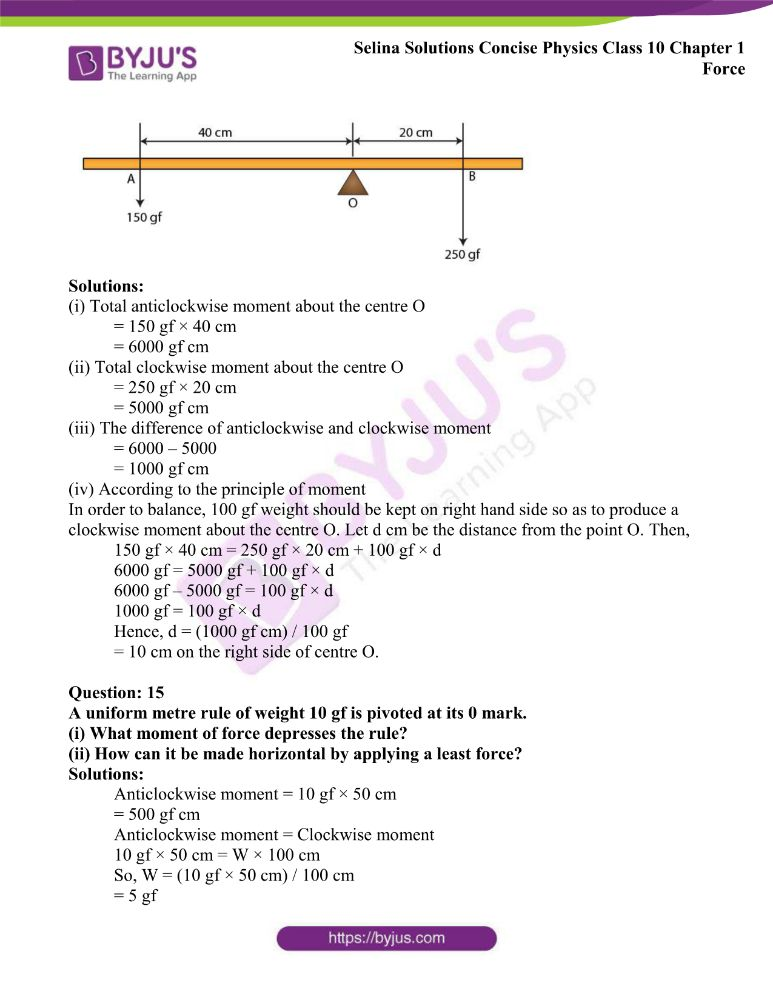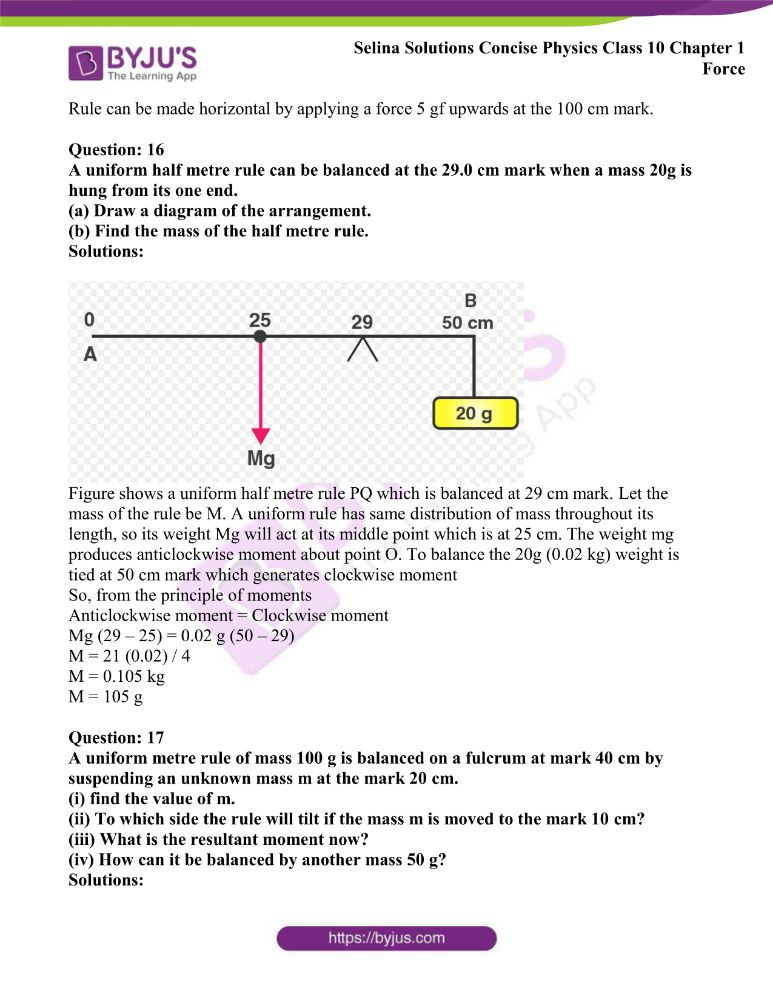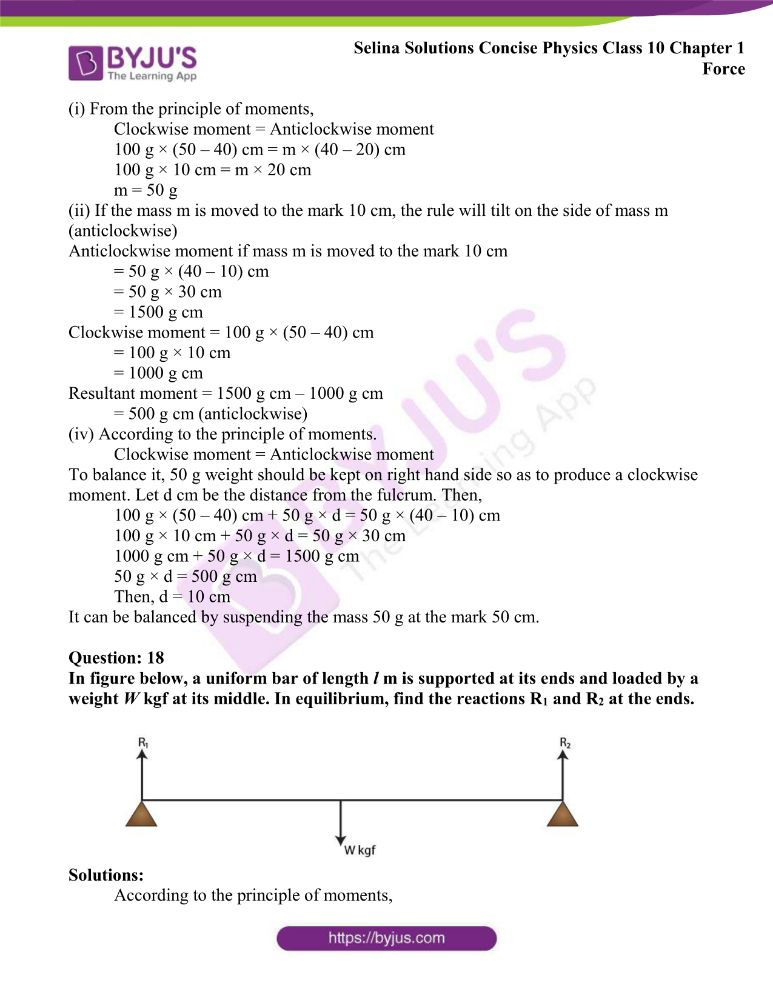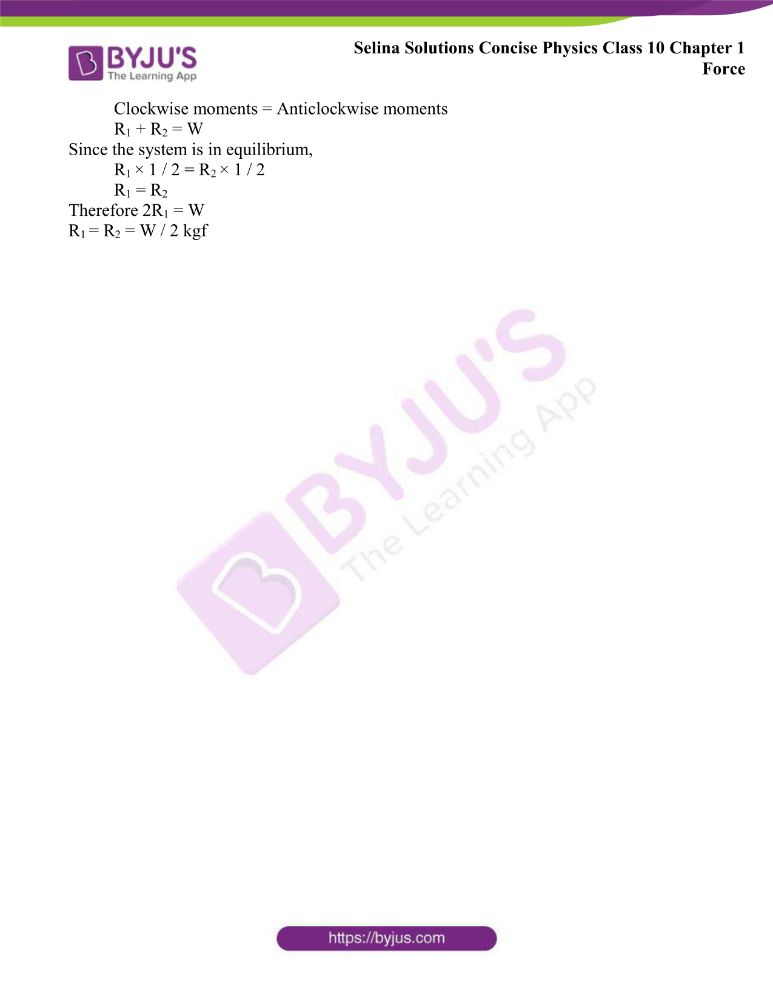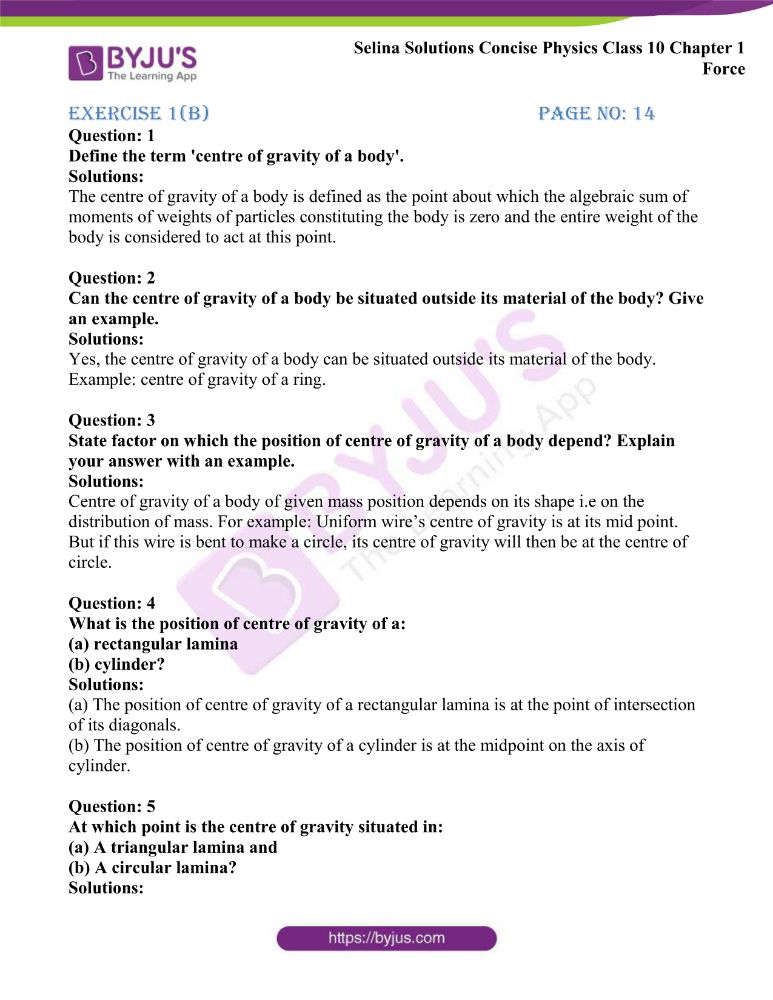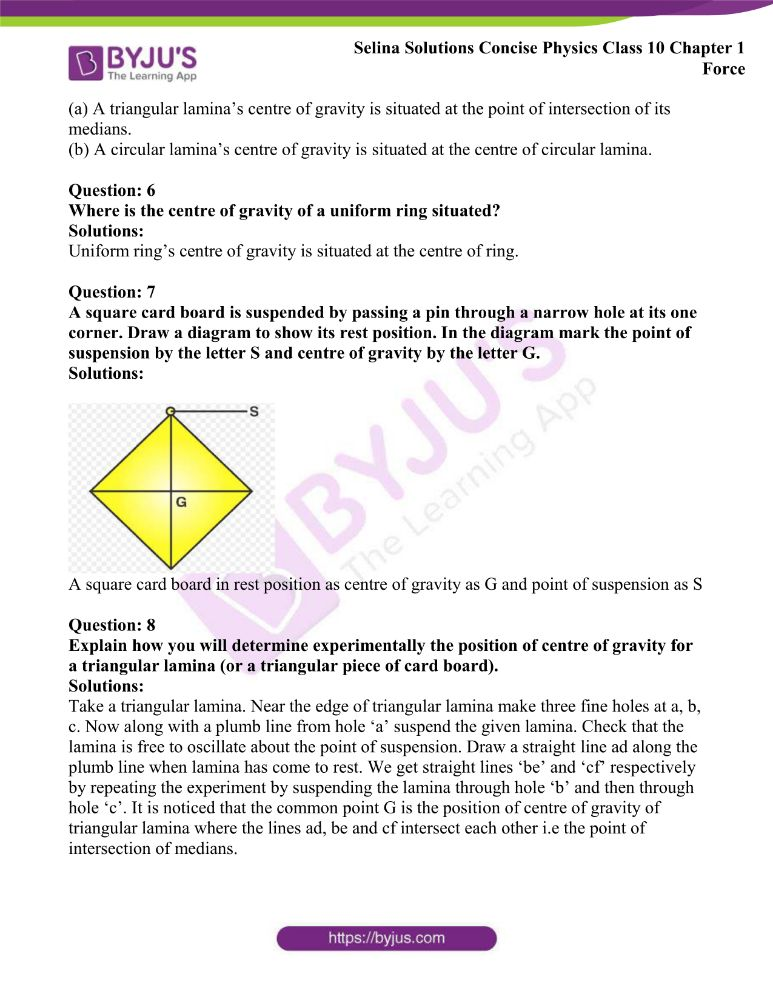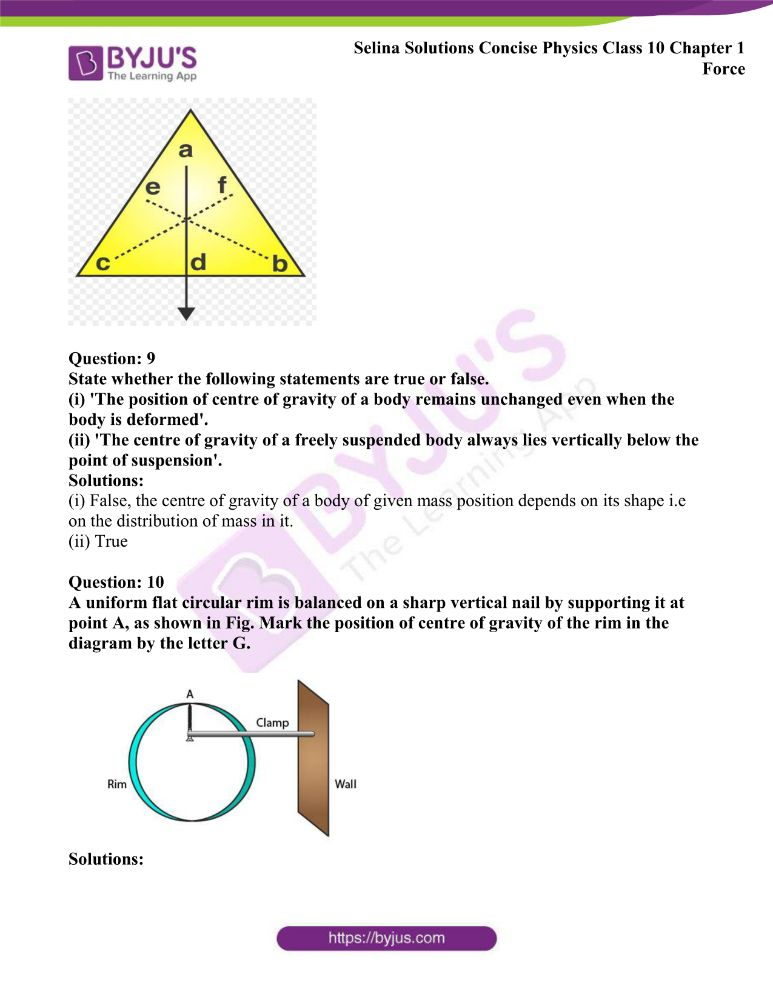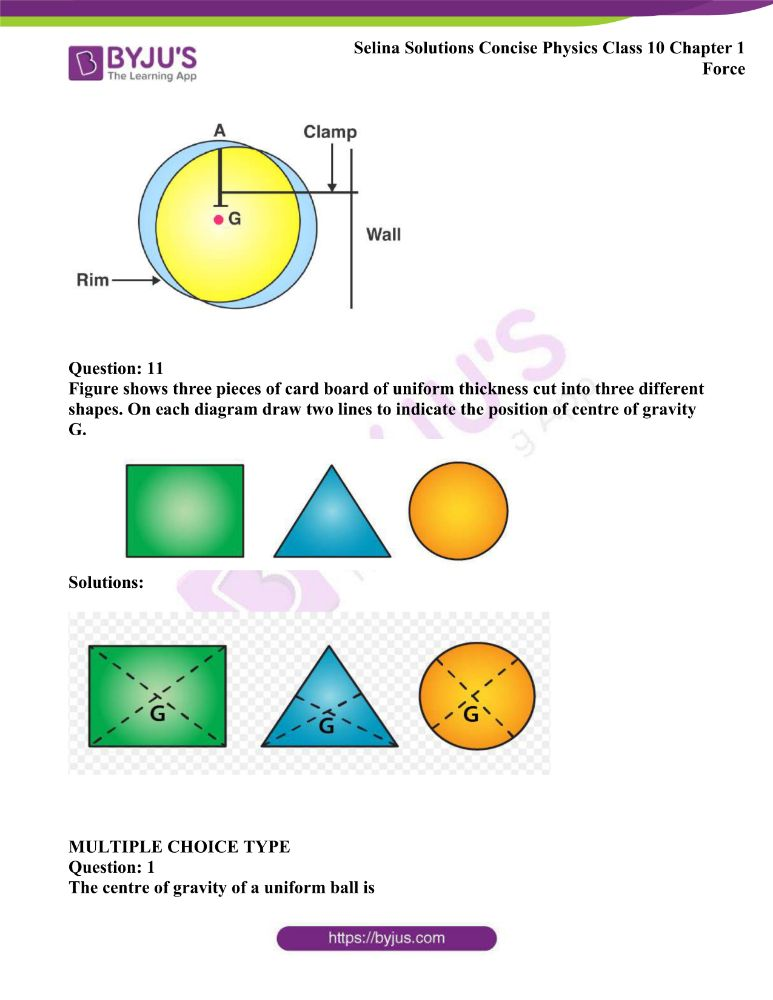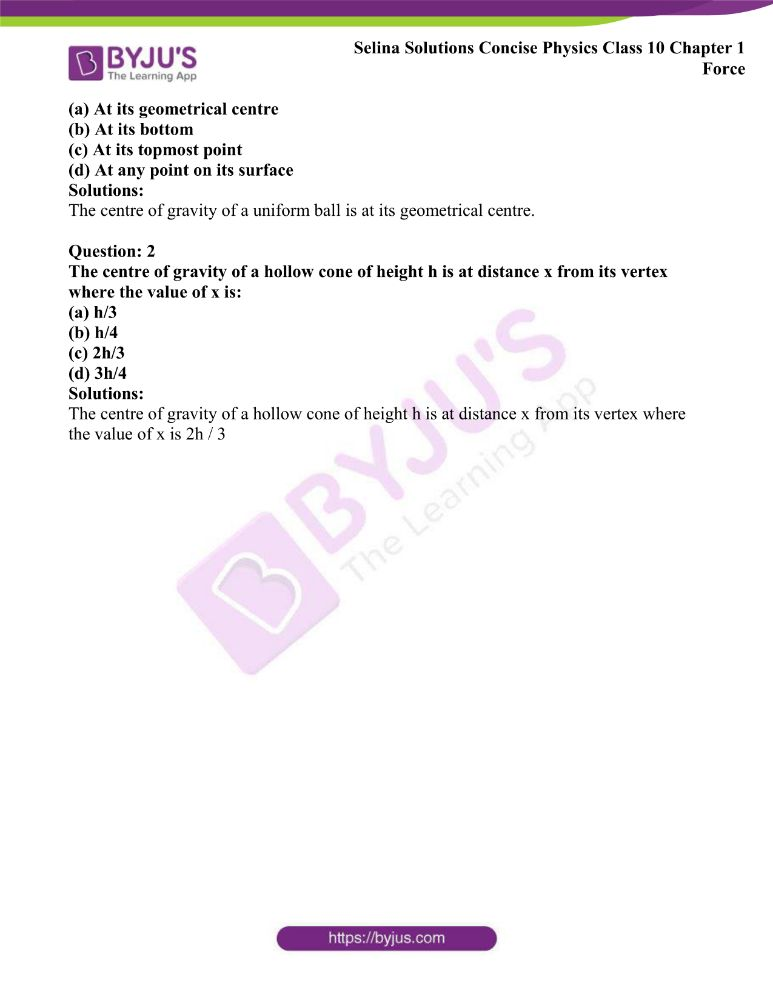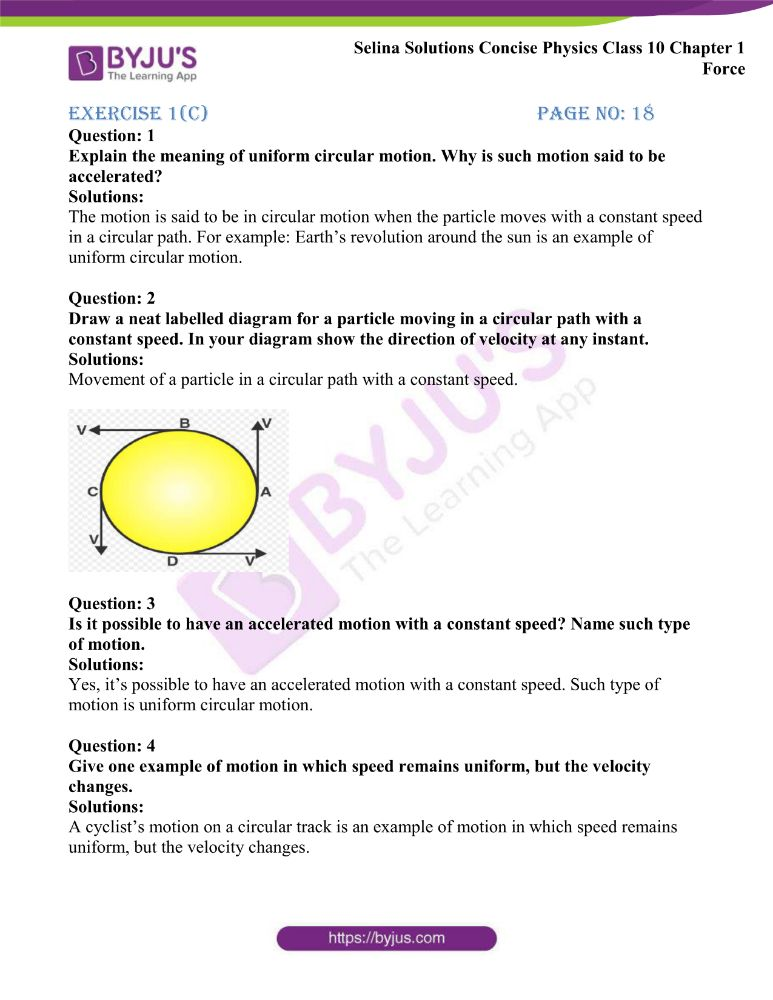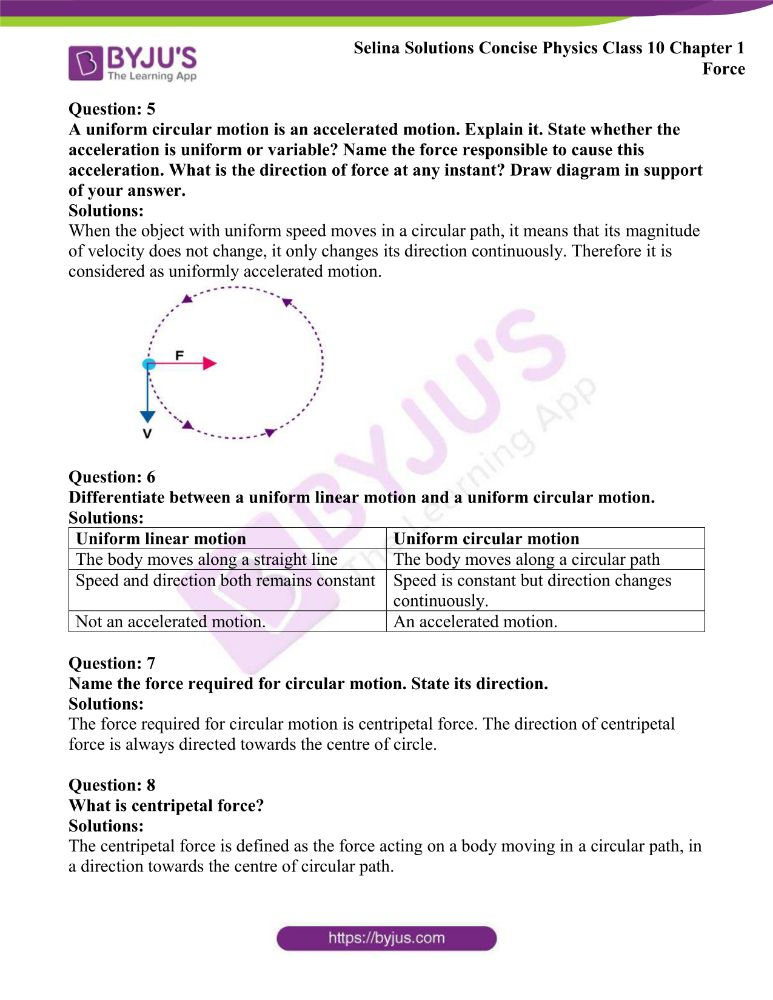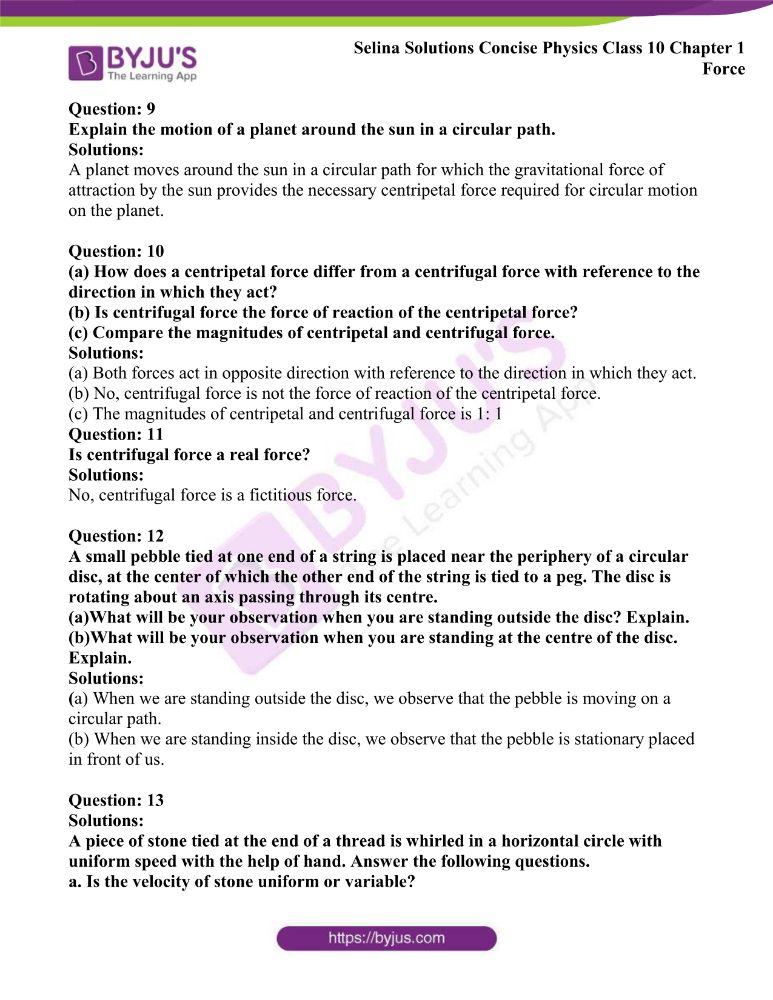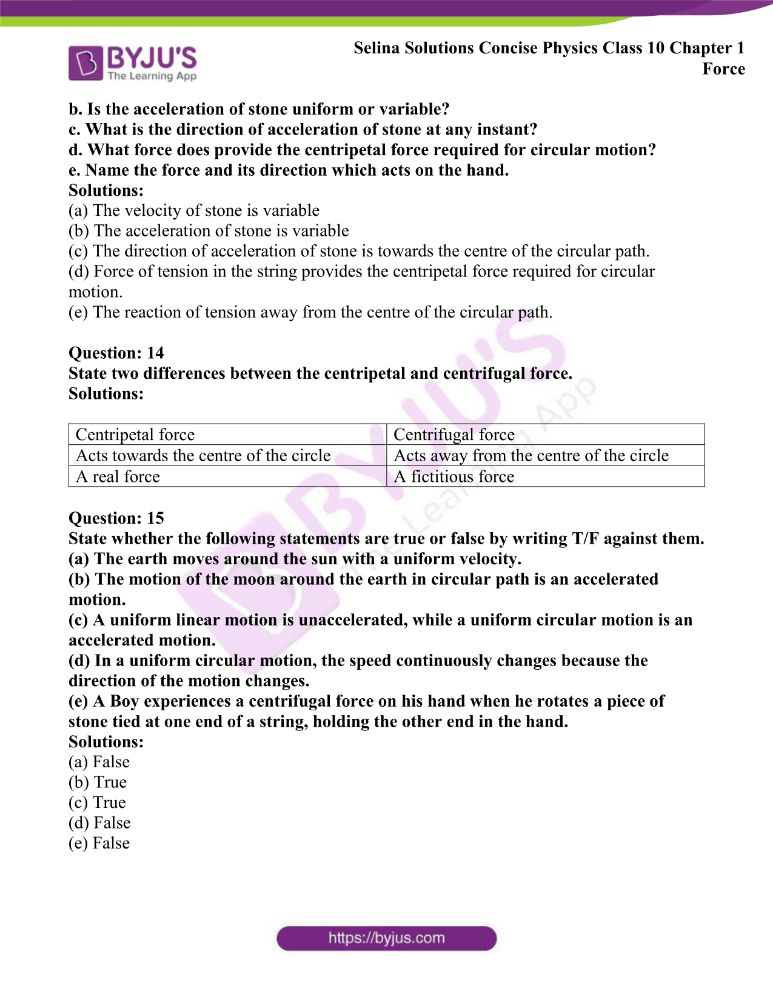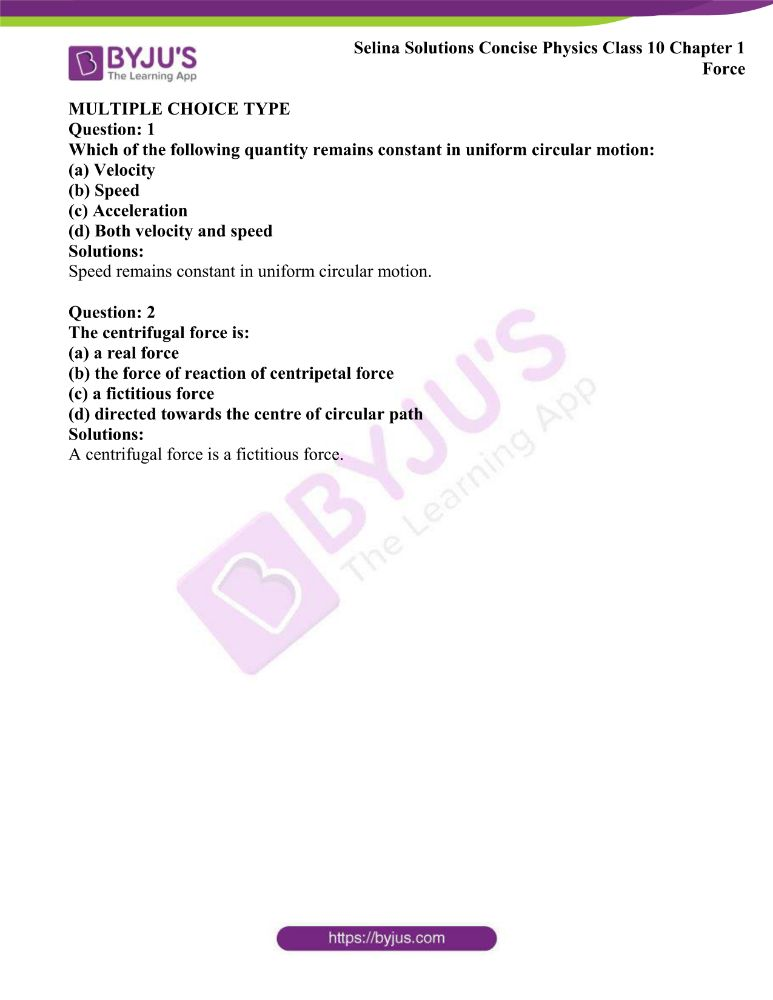### Access Answers of Physics Selina Solutions Concise Physics Chapter 1- Force

Exercise 1(A) Page no: 9

Question: 1

State the condition when applying a force, the body has:

(a)the translational motion,

(b)the rotational motion.

Solutions:

(a) Translational motion is produced when the body is free to move.

(b) Rotational motion is produced when the body is pivoted at a point.

Question: 2

Define the moment of force and state its S.I. unit.

Solutions:

The moment of force is equal to the product of the magnitude of the force and the perpendicular distance of the line of action of force from the axis of rotation.

The S.I unit of moment of force is Newton × meter

= Newton meter (Nm)

Question: 3

State whether the moment of force is a scalar or vector quantity?

Solutions:

The moment of force is a vector quantity.

Question: 4

State two factors affecting the turning effect of a force.

Solutions:

The following are the two factors on which moment of the force about a point depends

(a) The magnitude of the force applied and,

(b) The distance of the line of action of the force from the axis of rotation

Question: 5

When does a body rotate? State one way to change the direction of rotation of a body. Give a suitable example to explain your answer.

Solutions:

The force applied on the body at a suitable point rotates the body about the axis passing through the pivoted point, when the body is pivoted at a point.

By changing the point of application of force, the direction of rotation can be changed. Figures given below shows the anticlockwise and clockwise moments produced in a disc pivoted at its centre by changing the point of application of force F from A to B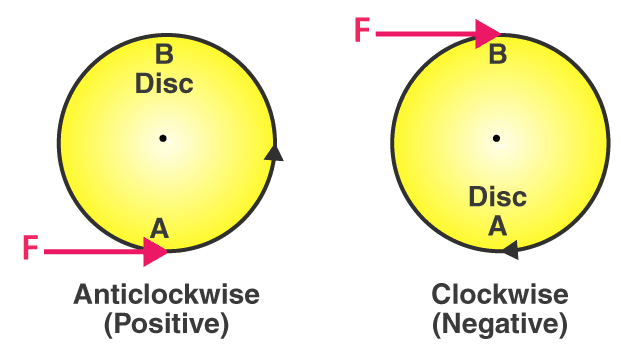Question: 6

Write the expression for the moment of force about a given axis.

Solutions:

The expression for the moment of force is given by

Moment of a force about a given axis = Force × perpendicular distance of force from the axis of rotation.

Question: 7

What do you understand by the clockwise and anticlockwise moment of force? When is it taken positive?

Solutions:

If the effect on the body is to turn it anticlockwise, a moment of force is called the anticlockwise moment and it is taken as positive, while if the effect on the body is to turn it clockwise, a moment of force is called the clockwise moment and it is taken as negative.

Question: 8

State one way to reduce the moment of a given force about a given axis of rotation.

Solutions:

The moment of force depends on the perpendicular distance of the line of action of force from the axis of rotation. The moment of a given force reduces by decreasing the perpendicular distance from the axis.

Question: 9

State one way to obtain a greater moment of a force about a given axis of rotation.

Solutions:

Moment of force is equal to the product of force and the perpendicular distance of force from the axis of rotation. By increasing the distance from the axis of rotation where the force would act is one of the ways to increase the moment of a force about a given axis of rotation.

Question: 10

Why is it easier to open a door by applying the force at the free end of it?

Solutions:

Larger the perpendicular distance, less force is needed to turn the body. Hence it is easier to open a door by applying the force at the free end of it.

Question: 11

The stone of hand flour grinder is provided with a handle near its rim. Give a reason.

Solutions:

The stone of hand flour grinder is provided with a handle near its rim because by applying small force at the handle it can be rotated easily about the iron pivot at its centre.

Question: 12

It is easier to turn the steering wheel of a large diameter than that of a small diameter. Give reason.

Solutions:

To turn the steering wheel of a large diameter needs less force which is at a large distance from the centre of the rim. So it is easier to turn the steering wheel of a large diameter than that of a small diameter.

Question: 13

A spanner (or wrench) has a long handle. Why?

Solutions:

The long handle of spanner produces a larger turning moment by which nut can easily be turned with a less force. So the spanner has a long handle.

Question: 14

A jackscrew is provided with a long arm. Explain why?

Solutions:

A long arm of a jackscrew which is used to lift a heavy load like a vehicle will help to apply less effort which is required to rotate it to raise or lower the jack. Hence the jackscrew has a long arm.

Question: 15

A, B and C are the three forces each of magnitude 4N acting in the plane of the paper as shown in Figure. The point O lies in the same plane.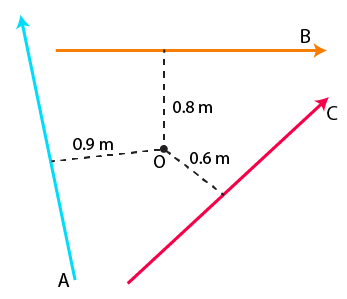(i) Which force has the least moment about O? Give a reason.

(ii) Which force has the greatest moment about O? Give a reason.

(iii) Name the forces producing

(a) clockwise and

(b) anticlockwise moments.

(iv)What is the resultant torque about the point O?

Solutions:

(i) As we know that,

Moment of force = Force × Perpendicular distance

Since vector C perpendicular distance is least from the point O

So, vector C will have the least moment about O.

(ii) As we know that,

Moment of force = Force × Perpendicular distance

Since vector A perpendicular distance is greatest from the point O

So, vector A will have greatest moment about O.

(iii) (a) Clockwise moments are produced by vectors A and B.

Explanation: If the turning effect on the body is clockwise, the moment of force is called clockwise moment and it is negative.

(b) Anticlockwise moment is produced by vector C

Explanation: If the turning effect on the body is anticlockwise, the moment of force is called anticlockwise moment and it is positive.

(iv) Sum of torques due to vectors A, B and C = Resultant torque about point O

So,

Resultant torque about point O = – (4 × 0.9) – (4 × 0.8) + (4 × 0.6) Nm

= – 3.6 – 3.2 + 2.4

= – 6.8 + 2.4

= – 4.4 Nm

Here the negative sign indicates that the resultant torque is in clockwise direction.

Question: 16

The adjacent diagram shows a heavy roller, with its axle at O, which is to be raised on a pavement XY. If there is friction between the roller and pavement, show by an arrow on the diagram the point of application and the direction of force to be applied.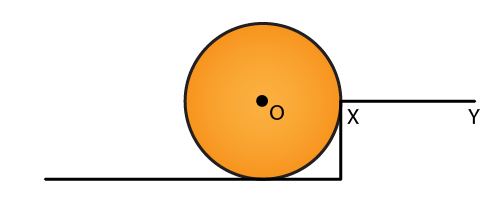Solutions: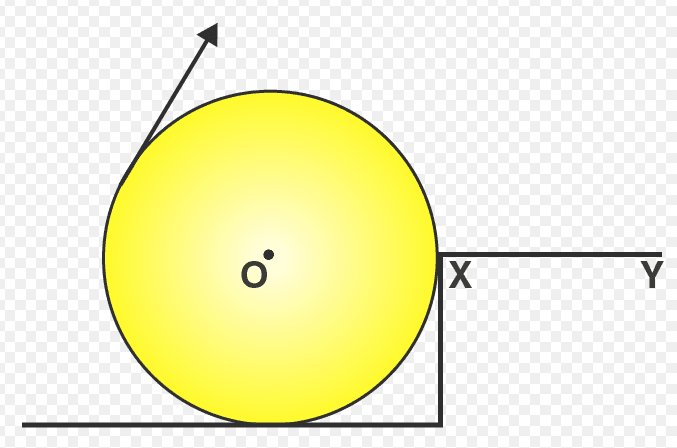Force F should be applied in the direction as shown in the above figure.

Question: 17

A body is acted upon by two forces each of magnitude F, but in opposite directions. State the effect of the forces if

(a)both forces act at the same point of the body.

(b)the two forces act at two different points of the body at a separation r.

Solutions:

(a) The resultant force acting on the body = 0

F – F = 0

(b) The forces tend to rotate the body between two forces about the midpoint.

Moment of forces = F × r

Fr

Question: 18

Draw a neat labelled diagram to show the direction of two forces acting on a body to produce rotation in it. Also mark the point O about which the rotation takes place.

Solution: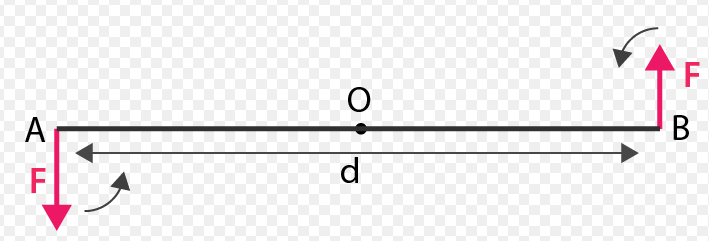At A and B, two forces each of magnitude F are applied, which are equal and opposite forces. By observing the above figure it is clear that the two forces rotate the bar in an anticlockwise direction.

Question: 19

What do you understand by the term couple? State its effect. Give two examples in our daily life where a couple is applied to turn a body.

Solutions:

Two forces not acting along the same line, which are equal and opposite parallel forces, form a couple. A couple is always required to produce the rotation.

Examples: turning a key in the hole of a lock and turning the steering wheel of a car.

Question: 20

Define the moment of a couple. Write its S.I unit.

Solutions:

Moment of the couple is equal to the product of both force and the perpendicular distance between the two forces.

The S.I unit of moment of couple is Nm

Question: 21

Prove that

Moment of couple = Force x Couple arm.

Solutions: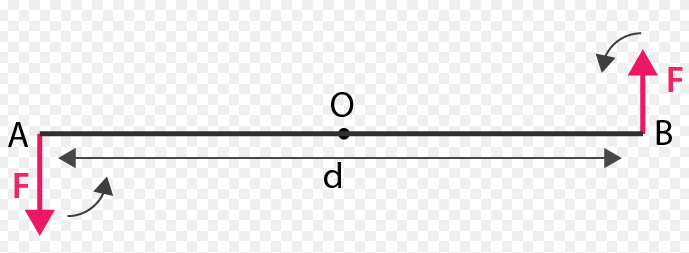At A and B two forces, each of magnitude F is applied, which are equal and opposite forces. The two forces rotate the bar in anticlockwise direction. AB is the perpendicular distance between two forces which is called the coupling arm.

Moment of force F at the end A

= F × OA (anticlockwise)

Moment of force F at the end B

= F × OB (anticlockwise)

Total moment of couple = F × OA + F × OB

= F × (OA + OB)

= F × AB

= F × d (anticlockwise)

= Either force × perpendicular distance between the two forces or couple arm

Hence, Moment of couple = Force × Couple arm

Question: 22

What do you mean by equilibrium of a body?

Solutions:

When a number of forces acting on a body produce no change in its state of rest or of linear or rotational motion, the body is said to be in equilibrium.

Question: 23

State the condition when a body is in (i) static, (ii) dynamic equilibrium. Give one example each of static and dynamic equilibrium.

Solutions:

(i) Static equilibrium is defined as when a body remains in the state of rest under the influence of several forces, the body is said to be in static equilibrium.

Example: A book lying on a table is in static equilibrium

(ii) Dynamic equilibrium is defined as when a body remains in the same state of motion under the influence of several forces, the body is said to be in dynamic equilibrium

Example: A rain drop reaches the earth surface with a constant velocity.

Question: 24

State two conditions for a body, acted upon by several forces to be in equilibrium.

Solutions:

The two conditions for a body to be in equilibrium are

(i) The resultant of all the forces acting on a body must be zero.

(ii) The algebraic sum of moments about the point of rotation of all the forces acting on the body should be zero.

Question: 25

State the principle of moments. Name one device based on it.

Solutions:

According to the principle of moments, if the algebraic sum of moments about the axis of rotation of all the forces acting on the body is zero, then the body is said to be in equilibrium. A beam balance is the device which works on the principle of moments.

Question: 26

Describe a simple experiment to verify the principle of moments, if you are supplied with a metre rule, a fulcrum and two springs with slotted weights.

Solutions:

Suspend a metre rule horizontally from a fixed support by means of a strong thread at point O as shown. Now with some slotted weights W1 and W2 suspend two spring balances on either side of the thread. The metre rule may tilt to one side. Now adjust the two spring balance distances from the support by keeping one at A and the other at B so that the scale again becomes horizontal.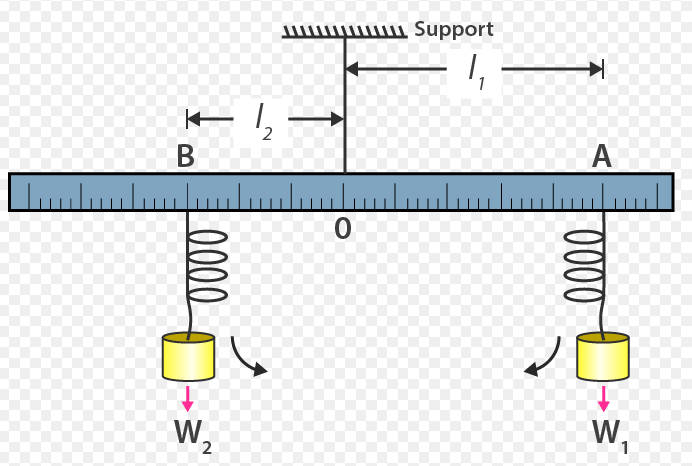Let the W1 at a distance OA = l1 be the weight suspended on the right side of thread from the spring balance at A, while W2 at a distance OB = l2 be the weight suspended on the left side of thread from the spring balance at B.

The weight W1 tends to turn the scale clockwise and the weight W2 tends to turn the scale anticlockwise.

Clockwise moment = W1 × l1

Anticlockwise moment = W2 × l2

In equilibrium, when the scale is horizontal, it is found that

Clockwise moment = Anticlockwise moment

Hence, W1l1 = W2l2

This verifies the principle of moments.

Question: 27

Complete the following sentences:

(i) The S.I. unit of moment of force is _________.

(ii) In equilibrium algebraic sum of moments of all forces about the point of rotation is ______________.

(iii) In a beam balance when the beam is balanced in a horizontal position, it is in ____________equilibrium.

(iv) The moon revolving around the earth is in ____________ equilibrium.

Solutions:

(i) The S.I. unit of moment of force is Newton metre (Nm)

(ii) In equilibrium algebraic sum of moments of all forces about the point of rotation is zero.

(iii) In beam balance when the beam is balanced in a horizontal position, it is in static equilibrium.

(iv) The moon revolving around the earth is in dynamic equilibrium.

MULTIPLE CHOICE TYPE

1. The moment of a force about a given axis depends:

(a) only on the magnitude of force

(b) only on the perpendicular distance of force from the axis

(c) neither on the force nor on the perpendicular distance of force from the axis

(d) both, on the force and its perpendicular distance from the axis.

Solution:

The moment of the force about a given axis depends on both, on the force and its perpendicular distance from the axis.

2. A body is acted upon by two unequal forces in opposite directions, but not in the same line. The effect is that:

(a) the body will have only the rotational motion

(b) the body will have only the translational motion

(c) the body will have neither the rotational motion nor the translational motion

(d) the body will have rotational as well as translational motion.

Solution:

A body is acted upon by two unequal forces in opposite directions, but not in the same line. The effect is that the body will have rotational as well as translational motion.

NUMERICALS

Question: 1

The moment of a force of 10N about a fixed point O is 5Nm. Calculate the distance of the point O from the line of action of the force.

Solutions:

We know that,

Moment of Force = Force × Perpendicular distance from the point O

= F × r

So, substituting the values given in the question, we get,

5Nm = 10r

r = 5 / 10

r = 0.5 m

Question: 2

A nut is opened by a wrench of length 10cm. If the least force required is 5.0N, find the moment of force needed to turn the nut.

Solutions:

Given

r = 10 cm

= 0.1 m

Required least force = 5 N

Moment of force = F × r

Substituting the values of F and r, we get

= 5 × 0.1

= 0.5 Nm

Question: 3

A wheel of diameter 2m is shown with the axle at O. A force F = 2N is applied at B in the direction shown in the figure. Calculate the moment of force about

(i) the centre O, and

(ii) the point A.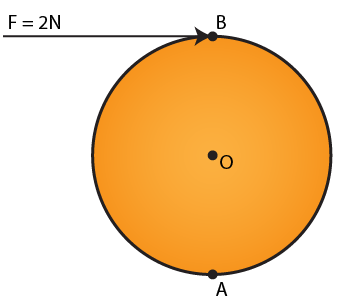Solutions:

Given

F = 2N

D = 2m

Since the diameter is 2m. So, the perpendicular distance between B and O is 1m

(i) Moment of force at the centre O

= F × r

= 2 × 1

= 2 Nm

– which is clockwise

(ii) Moment of force at point A

= F × r

= 2 × 2

= 4 Nm

– which is clockwise

Question: 4

The diagram shows two forces F1= 5N and F= 3N acting at points A and B of a rod pivoted at a point O, such that OA = 2m and OB = 4m

Calculate:

(i) the moment of force F1 about O.

(ii) the moment of force Fabout O.

(iii) total moment of the two forces about O.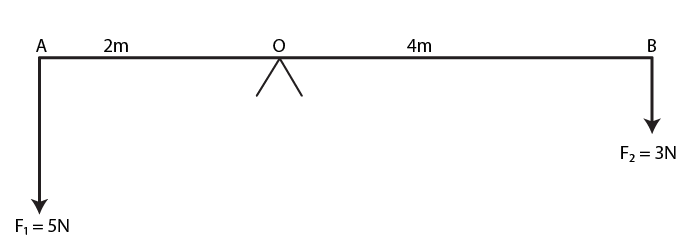Solutions:

Given

OA = 2m and OB = 4m

(i) Moment of force F1 = 5N about the point O at A

= F1 × OA

= 5 × 2

= 10Nm (anticlockwise)

(ii) Moment of force F2 = 3N about the point O at B

= F2 × OB

= 3 × 4

= 12 Nm (clockwise)

(iii) Total moment of forces about the midpoint O

= 12 – 10

= 2Nm (clockwise)

Question: 5

Two forces each of magnitude 10N act vertically upwards and downwards respectively at the two ends A and B of a uniform rod of length 4m which is pivoted at its mid-point O as shown. Determine the magnitude of the resultant moment of forces about the pivot O.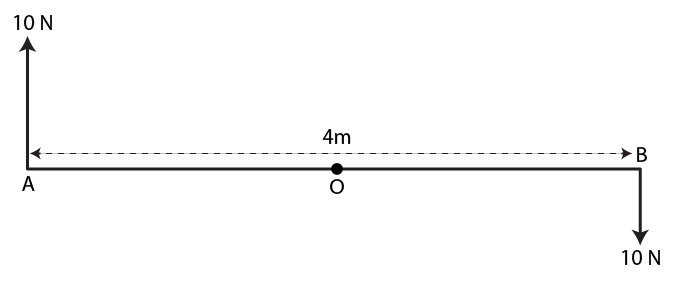Solutions:

Given

AB = 4m So, OA = 2m

Moment of force (F) = 10N about the O at point A

= F × OA

= 10 × 2

= 20 Nm (clockwise)

Moment of force (F) = 10N about the O at point B

= F × OB

= 10 × 2

= 20 Nm (clockwise)

Total moment of forces about the centre O

= 20 + 20

= 40 Nm (clockwise)

Question: 6

Figure shows two forces each of magnitude 10N acting at the points A and B at a separation of 50 cm, in opposite directions. Calculate the resultant moment of the two forces about the point (i) A, (ii) B and (iii) O, situated exactly at the middle of the two forces.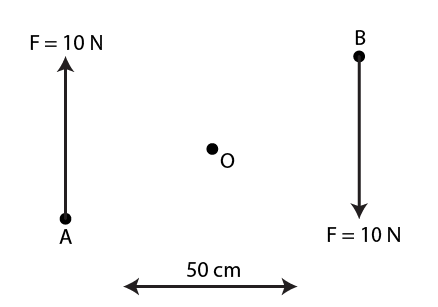Solutions:

(i) Perpendicular distance of point A from the force F = 10 N at B is 0.5m, when it is zero from the force F = 10N at point A

So, moment of force about A is

= 10N × 0.5Nm

= 5Nm (clockwise)

(ii) Perpendicular distance of point B from the force F = 10N at A is 0.5m, when it is zero from the force F = 10N at point B

So, moment of force about B is

= 10N × 0.5m

= 5Nm (clockwise)

(iii) Perpendicular distance of point O from either of the forces F = 10N is 0.25 m

Moment of force F = 10N at point A about O

= 10N × 0.25m

= 2.5Nm (clockwise)

Moment of force F = 10N at point B about O

= 10N × 0.25m

= 2.5Nm (clockwise)

∴ Total moment of both the forces about point O

= 0.25 + 0.25

= 5Nm (clockwise)

Question: 7

A steering wheel of diameter 0.5m is rotated anti-clockwise by applying two forces each of magnitude 5N. Draw a diagram to show the application of forces and calculate the moment of forces applied.

Solutions: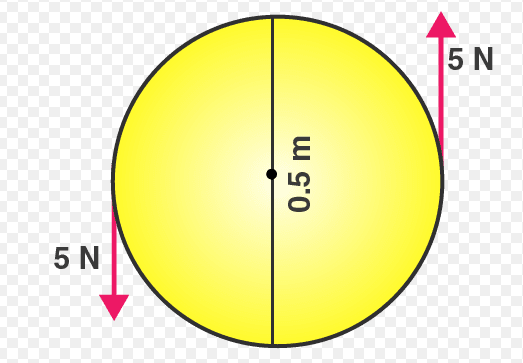Moment of couple = either force × couple arm

= 5N × 0.5m

= 2.5 Nm

Question: 8

A uniform metre rule is pivoted at its mid-point. A weight of 50 gf is suspended at one end of it. Where a weight of 100gf should be suspended to keep the rule horizontal?

Solutions:

Let the 50 gf weight produce anticlockwise moment about the middle point i.e at 50 cm

Let the weight of 100 gf produce a clockwise moment about the middle point and d cm be the distance from the middle. So,

According to the principle of moments.

Anticlockwise moment = Clockwise moment

50 gf × 50 cm = 100 gf × d

Then d = (50 × 50) / 100

= 25 cm

A weight of 100 gf is suspended at a distance 25 cm from the other end to keep the rule horizontal.

Question: 9

A uniform metre rule balances horizontally on a knife edge placed at the 58 cm mark when a weight of 20gf is suspended from one end.

(i)Draw a diagram of the arrangement.

(ii)What is the weight of the rule?

Solutions:

(i) Weight of rule produces an anticlockwise moment about the knife edge O. In order to balance 20 gf should be suspended at the end B to produce clockwise moment about the knife edge O

Figure

(ii) According to the principle of moments,

Anticlockwise moment = Clockwise moment

W × (58 – 50)

= 20 gf × (100 – 58)

W × 8 = 20 gf × 42

W = (20 gf × 42) / 8

= 105 gf

Question: 10

The diagram shows a uniform bar supported at the middle point O. A weight of 40 gf is placed at a distance 40cm to the left of the point O. How can you balance the bar with a weight of 80 gf?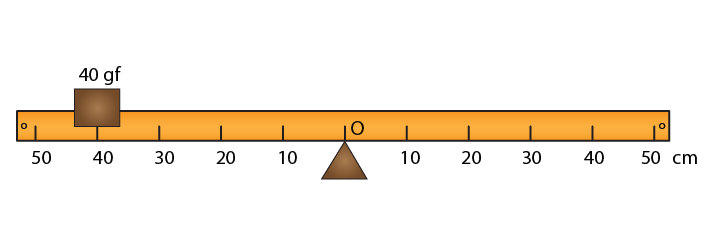Solutions:

Anticlockwise moment = 40 gf × 40 cm

Clockwise moment = 80 gf × d cm

According to principle of moments,

Anticlockwise moment = Clockwise moment

40 gf × 40 cm = 80 gf × d

Hence d = (40 gf × 40) / 80

= 20 cm to the right of point O

We can balance the bar by placing the weight of 80 gf at a distance 20 cm to the right of point O.

Question: 11

Figure shows a uniform metre rule placed on a fulcrum at its mid-point O and having a weight 40gf at the 10 cm mark and a weight of 20 gf at the 90 cm mark.

(i) Is the metre rule in equilibrium? If not, how will the rule turn?

(ii) How can the rule be brought in equilibrium by using an additional weight of 40gf?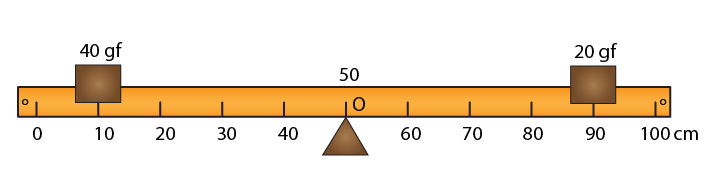Solutions:

(i)Anticlockwise moment = 40 gf × (50 – 10) cm

= 40 gf × 40 cm

= 1600 gf × cm

Clockwise moment = 20 gf × (90 – 50)

= 20 gf × 40 cm

= 800 gf × cm

Anticlockwise moment is not equal to clockwise moment. So the metre rule is not in equilibrium and it will turn anticlockwise.

(ii) In order to balance 40 gf weight should be kept on right hand side so as to produce a clockwise moment about the middle point. Let d cm be the distance from the middle. Then,

Clockwise moment = 20 gf × 40 cm + 40 gf × d cm

According to the principle of moments,

Anticlockwise moment = Clockwise moment

40 gf × 40 cm = 20 gf × 40 cm + 40 gf × d cm

1600 = 800 + 40 gf × d cm

1600 – 800 = 40 gf × d cm

Hence d = (800 gf cm) / 40

d = 20 cm (on the other side)

The rule can be brought in equilibrium by placing the additional weight of 40 gf at the 70 cm mark.

Question: 12

When a boy weighing 20 kgf sits at one end of a 4m long see-saw, it gets depressed at its end. How can it be brought to the horizontal position by a man weighing 40 kgf.

Solutions:

According to the principle of moment,

Anticlockwise moment = Clockwise moment

20 kgf × 2 m = 40 kgf × d

So, d = (20kgf × 2m) / 40 kgf

= 1 m

It can be brought to the horizontal position if a man sits at a distance 1 m from the centre on the side opposite to the boy.

Question: 13

A physical balance has its arms of length 60 cm and 40 cm. What weight kept on a pan of longer arm will balance an object of weight 100 gf kept on other pan?

Solutions:

According to the principle of moments,

Anticlockwise moment = Clockwise moment

100 gf × 40 cm = W × 60 cm

Hence, weight on the longer pan,

W = (100 gf × 40 cm) / 60 cm

= 200 gf / 3

= 66.67 gf

Question: 14

The diagram shows a uniform metre rule weighing 100 gf, pivoted at its centre O. Two weights 150gf and 250gf hang from the point A and B respectively of the metre rule such that OA = 40 cm and OB = 20 cm. Calculate: (i) the total anticlockwise moment about O, (ii) the total clockwise moment about O, (iii) the difference of anticlockwise and clockwise moment, and (iv) the distance from O where a 100gf weight should be placed to balance the metre rule.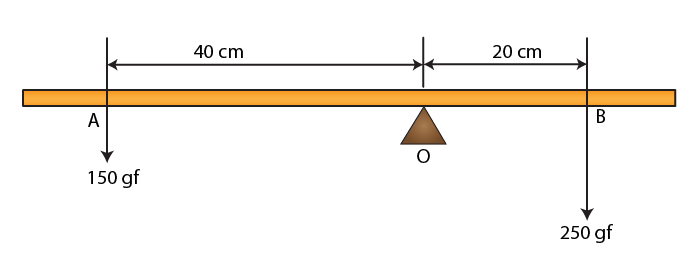Solutions:

(i) Total anticlockwise moment about the centre O

= 150 gf × 40 cm

= 6000 gf cm

(ii) Total clockwise moment about the centre O

= 250 gf × 20 cm

= 5000 gf cm

(iii) The difference of anticlockwise and clockwise moment

= 6000 – 5000

= 1000 gf cm

(iv) According to the principle of moment

In order to balance, 100 gf weight should be kept on the right-hand side so as to produce a clockwise moment about the centre O. Let d cm be the distance from the point O. Then,

150 gf × 40 cm = 250 gf × 20 cm + 100 gf × d

6000 gf = 5000 gf + 100 gf × d

6000 gf – 5000 gf = 100 gf × d

1000 gf = 100 gf × d

Hence, d = (1000 gf cm) / 100 gf

= 10 cm on the right side of centre O.

Question: 15

A uniform metre rule of weight 10 gf is pivoted at its 0 mark.

(i) What moment of force depresses the rule?

(ii) How can it be made horizontal by applying a least force?

Solutions:

Anticlockwise moment = 10 gf × 50 cm

= 500 gf cm

Anticlockwise moment = Clockwise moment

10 gf × 50 cm = W × 100 cm

So, W = (10 gf × 50 cm) / 100 cm

= 5 gf

Rule can be made horizontal by applying a force 5 gf upwards at the 100 cm mark.

Question: 16

A uniform half-metre rule can be balanced at the 29.0 cm mark when a mass 20g is hung from its one end.

(a) Draw a diagram of the arrangement.

(b) Find the mass of the half-metre rule.

Solutions: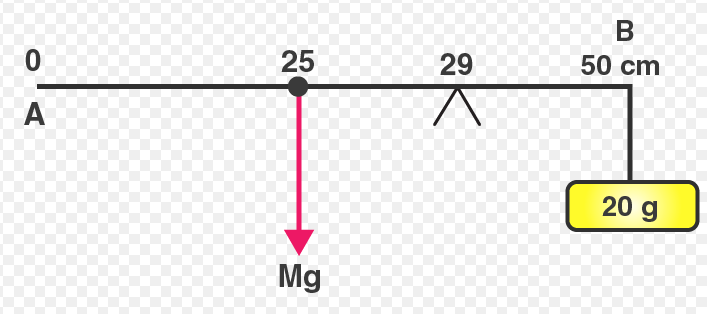Figure shows a uniform half-metre rule PQ which is balanced at 29 cm mark. Let the mass of the rule be M. A uniform rule has the same distribution of mass throughout its length, so its weight Mg will act at its middle point which is at 25 cm. The weight mg produces anticlockwise moment about point O. To balance the 20g (0.02 kg) weight is tied at 50 cm mark which generates clockwise moment

So, from the principle of moments

Anticlockwise moment = Clockwise moment

Mg (29 – 25) = 0.02 g (50 – 29)

M = 21 (0.02) / 4

M = 0.105 kg

M = 105 g

Question: 17

A uniform metre rule of mass 100 g is balanced on a fulcrum at mark 40 cm by suspending an unknown mass m at the mark 20 cm.

(i) find the value of m.

(ii) To which side the rule will tilt if the mass m is moved to the mark 10 cm?

(iii) What is the resultant moment now?

(iv) How can it be balanced by another mass 50 g?

Solutions:

(i) From the principle of moments,

Clockwise moment = Anticlockwise moment

100 g × (50 – 40) cm = m × (40 – 20) cm

100 g × 10 cm = m × 20 cm

m = 50 g

(ii) If the mass m is moved to the mark 10 cm, the rule will tilt on the side of mass m (anticlockwise)

Anticlockwise moment if mass m is moved to the mark 10 cm

= 50 g × (40 – 10) cm

= 50 g × 30 cm

= 1500 g cm

Clockwise moment = 100 g × (50 – 40) cm

= 100 g × 10 cm

= 1000 g cm

Resultant moment = 1500 g cm – 1000 g cm

= 500 g cm (anticlockwise)

(iv) According to the principle of moments.

Clockwise moment = Anticlockwise moment

To balance it, 50 g weight should be kept on right-hand side so as to produce a clockwise moment. Let d cm be the distance from the fulcrum. Then,

100 g × (50 – 40) cm + 50 g × d = 50 g × (40 – 10) cm

100 g × 10 cm + 50 g × d = 50 g × 30 cm

1000 g cm + 50 g × d = 1500 g cm

50 g × d = 500 g cm

Then, d = 10 cm

It can be balanced by suspending the mass 50 g at the mark 50 cm.

Question: 18

In figure below, a uniform bar of length l m is supported at its ends and loaded by a weight W kgf at its middle. In equilibrium, find the reactions R1 and R2 at the ends.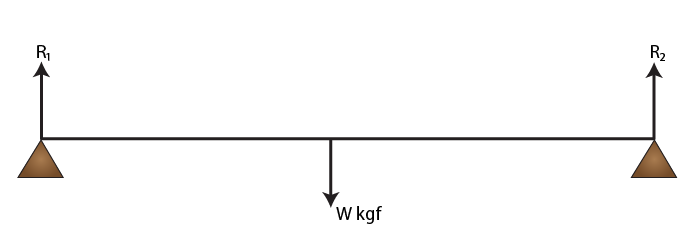Solutions:

According to the principle of moments,

Clockwise moments = Anticlockwise moments

R1 + R2 = W

Since the system is in equilibrium,

R1 × 1 / 2 = R2 × 1 / 2

R1 = R2

Therefore 2R1 = W

R1 = R2 = W / 2 kgf

Exercise 1(B) page no: 14

Question: 1

Define the term ‘centre of gravity of a body’.

Solutions:

The centre of gravity of a body is defined as the point about which the algebraic sum of moments of weights of particles constituting the body is zero and the entire weight of the body is considered to act at this point.

Question: 2

Can the centre of gravity of a body be situated outside its material of the body? Give an example.

Solutions:

Yes, the centre of gravity of a body can be situated outside its material of the body.

Example: centre of gravity of a ring.

Question: 3

State factor on which the position of the centre of gravity of a body depend? Explain your answer with an example.

Solutions:

Centre of gravity of a body of given mass position depends on its shape i.e on the distribution of mass. For example: Uniform wire’s centre of gravity is at its midpoint. But if this wire is bent to make a circle, its centre of gravity will then be at the centre of the circle.

Question: 4

What is the position of the centre of gravity of a:

(a) rectangular lamina

(b) cylinder?

Solutions:

(a) The position of centre of gravity of a rectangular lamina is at the point of intersection of its diagonals.

(b) The position of centre of gravity of a cylinder is at the midpoint on the axis of the cylinder.

Question: 5

At which point is the centre of gravity situated in:

(a) A triangular lamina and

(b) A circular lamina?

Solutions:

(a) A triangular lamina’s centre of gravity is situated at the point of intersection of its medians.

(b) A circular lamina’s centre of gravity is situated at the centre of the circular lamina.

Question: 6

Where is the centre of gravity of a uniform ring situated?

Solutions:

Uniform ring’s centre of gravity is situated at the centre of the ring.

Question: 7

A square card board is suspended by passing a pin through a narrow hole at its one corner. Draw a diagram to show its rest position. In the diagram mark the point of suspension by the letter S and centre of gravity by the letter G.

Solutions: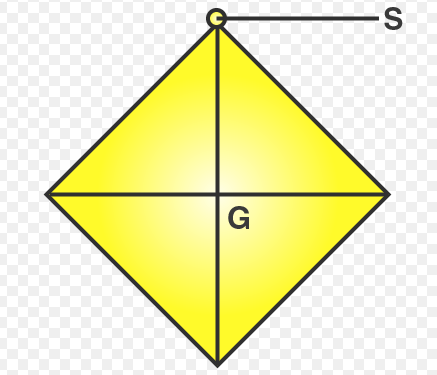A square cardboard in rest position as centre of gravity as G and point of suspension as S

Question: 8

Explain how you will determine experimentally the position of the centre of gravity for a triangular lamina (or a triangular piece of cardboard).

Solutions:

Take a triangular lamina. Near the edge of triangular lamina make three fine holes at a, b, c. Now along with a plumb line from hole ‘a’ suspend the given lamina. Check that the lamina is free to oscillate about the point of suspension. Draw a straight line ad along the plumb line when lamina has come to rest. We get straight lines ‘be’ and ‘cf’ respectively by repeating the experiment by suspending the lamina through-hole ‘b’ and then through hole ‘c’. It is noticed that the common point G is the position of the centre of gravity of triangular lamina where the lines ad, be and cf intersect each other i.e the point of intersection of medians.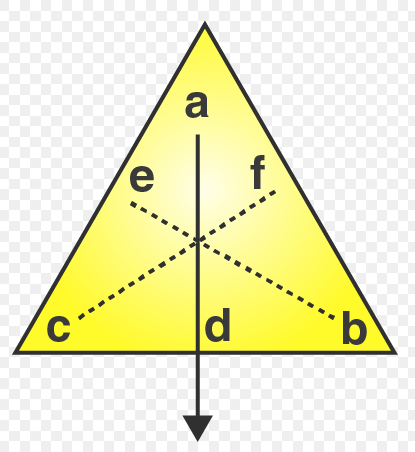Question: 9

State whether the following statements are true or false.

(i) ‘The position of the centre of gravity of a body remains unchanged even when the body is deformed’.

(ii) ‘The centre of gravity of a freely suspended body always lies vertically below the point of suspension’.

Solutions:

(i) False, the centre of gravity of a body of given mass position depends on its shape i.e on the distribution of mass in it.

(ii) True

Question: 10

A uniform flat circular rim is balanced on a sharp vertical nail by supporting it at point A, as shown in Fig. Mark the position of the centre of gravity of the rim in the diagram by the letter G.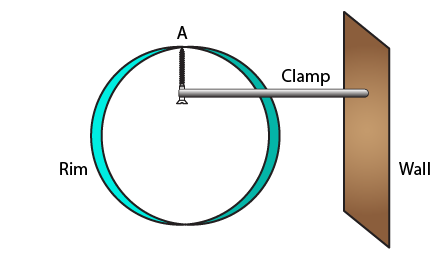Solutions: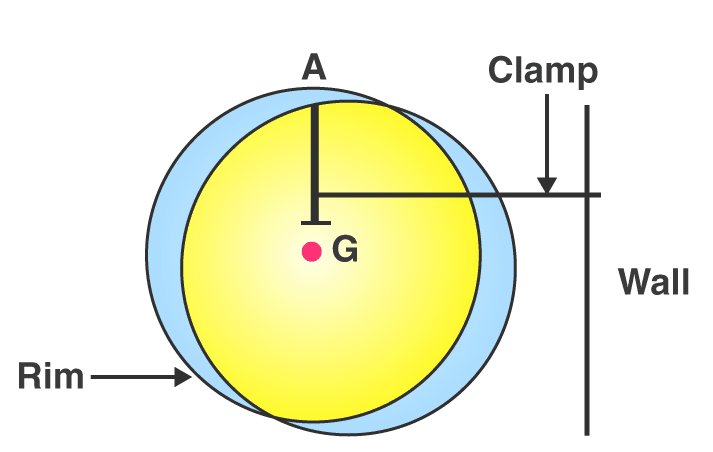Question: 11

Figure shows three pieces of cardboard of uniform thickness cut into three different shapes. On each diagram draw two lines to indicate the position of the centre of gravity G.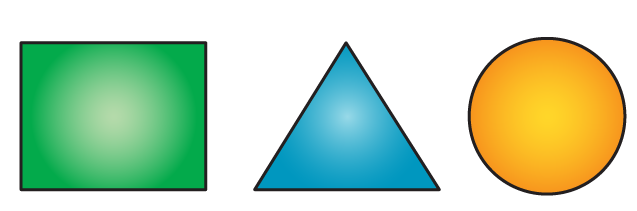Solutions: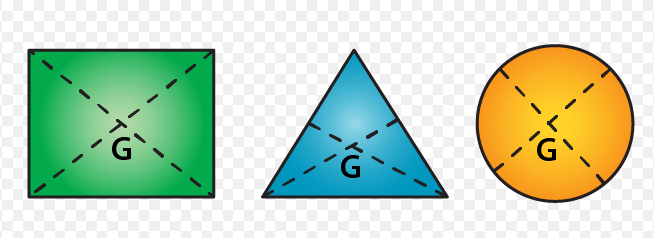MULTIPLE CHOICE TYPE

Question: 1

The centre of gravity of a uniform ball is

(a) At its geometrical centre

(b) At its bottom

(c) At its topmost point

(d) At any point on its surface

Solutions:

The centre of gravity of a uniform ball is at its geometrical centre.

Question: 2

The centre of gravity of a hollow cone of height h is at distance x from its vertex where the value of x is:

(a) h/3

(b) h/4

(c) 2h/3

(d) 3h/4

Solutions:

The centre of gravity of a hollow cone of height h is at distance x from its vertex where the value of x is 2h / 3

Exercise 1(c) page no: 18

Question: 1

Explain the meaning of uniform circular motion. Why is such motion said to be accelerated?

Solutions:

The motion is said to be in a circular motion when the particle moves with a constant speed in a circular path. For example: Earth’s revolution around the sun is an example of uniform circular motion.

Question: 2

Draw a neat labelled diagram for a particle moving in a circular path with a constant speed. In your diagram show the direction of velocity at any instant.

Solutions:

Movement of a particle in a circular path with a constant speed.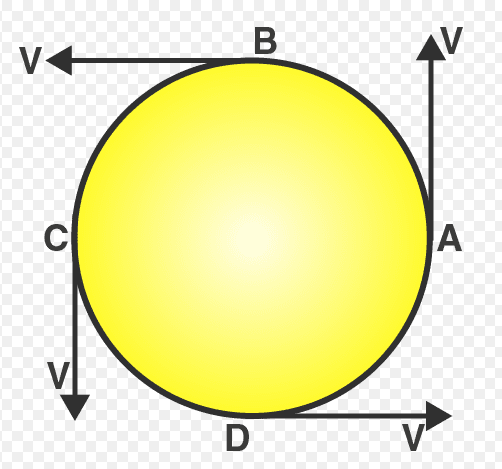Question: 3

Is it possible to have an accelerated motion with a constant speed? Name such type of motion.

Solutions:

Yes, it’s possible to have an accelerated motion with a constant speed. Such type of motion is uniform circular motion.

Question: 4

Give one example of motion in which speed remains uniform, but the velocity changes.

Solutions:

A cyclist’s motion on a circular track is an example of motion in which speed remains uniform, but the velocity changes.

Question: 5

A uniform circular motion is an accelerated motion. Explain it. State whether the acceleration is uniform or variable? Name the force responsible to cause this acceleration. What is the direction of force at any instant? Draw diagram in support of your answer.

Solutions:

When the object with uniform speed moves in a circular path, it means that its magnitude of velocity does not change, it only changes its direction continuously. Therefore it is considered as uniformly accelerated motion.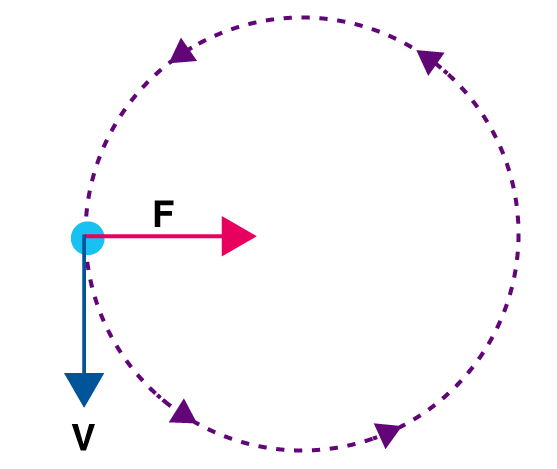Question: 6

Differentiate between a uniform linear motion and a uniform circular motion.

Solutions:

 Uniform linear motion Uniform circular motion The body moves along a straight line The body moves along a circular path Speed and direction both remains constant Speed is constant but direction changes continuously. Not an accelerated motion. An accelerated motion.

Question: 7

Name the force required for circular motion. State its direction.

Solutions:

The force required for circular motion is centripetal force. The direction of centripetal force is always directed towards the centre of the circle.

Question: 8

What is centripetal force?

Solutions:

The centripetal force is defined as the force acting on a body moving in a circular path, in a direction towards the centre of circular path.

Question: 9

Explain the motion of a planet around the sun in a circular path.

Solutions:

A planet moves around the sun in a circular path for which the gravitational force of attraction by the sun provides the necessary centripetal force required for circular motion on the planet.

Question: 10

(a) How does a centripetal force differ from a centrifugal force with reference to the direction in which they act?

(b) Is centrifugal force the force of reaction of the centripetal force?

(c) Compare the magnitudes of centripetal and centrifugal force.

Solutions:

(a) Both forces act in the opposite direction with reference to the direction in which they act.

(b) No, centrifugal force is not the force of reaction of the centripetal force.

(c) The magnitudes of centripetal and centrifugal force is 1: 1

Question: 11

Is centrifugal force a real force?

Solutions:

No, centrifugal force is a fictitious force.

Question: 12

A small pebble tied at one end of a string is placed near the periphery of a circular disc, at the centre of which the other end of the string is tied to a peg. The disc is rotating about an axis passing through its centre.

(a)What will be your observation when you are standing outside the disc? Explain.

(b)What will be your observation when you are standing at the centre of the disc. Explain.

Solutions:

(a) When we are standing outside the disc, we observe that the pebble is moving on a circular path.

(b) When we are standing inside the disc, we observe that the pebble is stationary placed in front of us.

Question: 13

Solutions:

A piece of stone tied at the end of a thread is whirled in a horizontal circle with uniform speed with the help of hand. Answer the following questions.

a. Is the velocity of stone uniform or variable?

b. Is the acceleration of stone uniform or variable?

c. What is the direction of acceleration of stone at any instant?

d. What force does provide the centripetal force required for circular motion?

e. Name the force and its direction which acts on the hand.

Solutions:

(a) The velocity of stone is variable

(b) The acceleration of stone is variable

(c) The direction of acceleration of stone is towards the centre of the circular path.

(d) Force of tension in the string provides the centripetal force required for circular motion.

(e) The reaction of tension away from the centre of the circular path.

Question: 14

State two differences between the centripetal and centrifugal force.

Solutions:

 Centripetal force Centrifugal force Acts towards the centre of the circle Acts away from the centre of the circle A real force A fictitious force

Question: 15

State whether the following statements are true or false by writing T/F against them.

(a) The earth moves around the sun with a uniform velocity.

(b) The motion of the moon around the earth in circular path is an accelerated motion.

(c) A uniform linear motion is unaccelerated, while a uniform circular motion is an accelerated motion.

(d) In a uniform circular motion, the speed continuously changes because the direction of the motion changes.

(e) A Boy experiences a centrifugal force on his hand when he rotates a piece of stone tied at one end of a string, holding the other end in the hand.

Solutions:

(a) False

(b) True

(c) True

(d) False

(e) False

MULTIPLE CHOICE TYPE

Question: 1

Which of the following quantity remains constant in uniform circular motion:

(a) Velocity

(b) Speed

(c) Acceleration

(d) Both velocity and speed

Solutions:

Speed remains constant in uniform circular motion.

Question: 2

The centrifugal force is:

(a) a real force

(b) the force of reaction of centripetal force

(c) a fictitious force

(d) directed towards the centre of the circular path

Solutions:

A centrifugal force is a fictitious force.

## Selina Solutions Concise Physics Class 10 Chapter 1- Force

ICSE Class 10 Physics Chapter 1 Force gives the students a clear understanding about the concepts translational and rotational motions, moment of a force or torque, couple, equilibrium bodies, principle of moments, centre of gravity, uniform circular motion, centripetal and centrifugal force. The chapter also enlightens students about simple numerical problems and its derivations to help students learn better. By referring to the topics included in this chapter students can easily answer the questions of the textbook. Also, they get a clear idea of how to answer the questions in accordance with the exam point of view. This helps the students to prepare well for exam preparation

List of subtopics covered in Concise Selina Solutions for Class 10 Physics Chapter 1 – Force

 Number Subtopic 1.1 Translational and rotational motions 1.2 Moment of a force or torque 1.3 Couple 1.4 Equilibrium of bodies 1.5 Principle of moments 1.6 Centre of gravity 1.7 Uniform circular motion 1.8 Centripetal and centrifugal force

List of Exercise

 Name of the exercise Page number 1(A) 9 1(B) 14 1(C) 18

### Key Features of Selina Solutions Concise Physics Class 10 Chapter 1 – Force

• Selina Solutions is the best study tool for students
• Solutions are provided comprehensively
• Numerical problems are provided with thorough explanations
• Solutions are developed according to the latest I.C.S.E syllabus

The given solutions are as per the 2019-20 Concise Selina textbook. The Selina Solutions for the academic year 2020-21 will be updated soon.

#### 1 Comment

1. Dr GNVerma

excellent material we are thankful to byjus